# 红黑树

## 前言## 红黑树与 2-3 树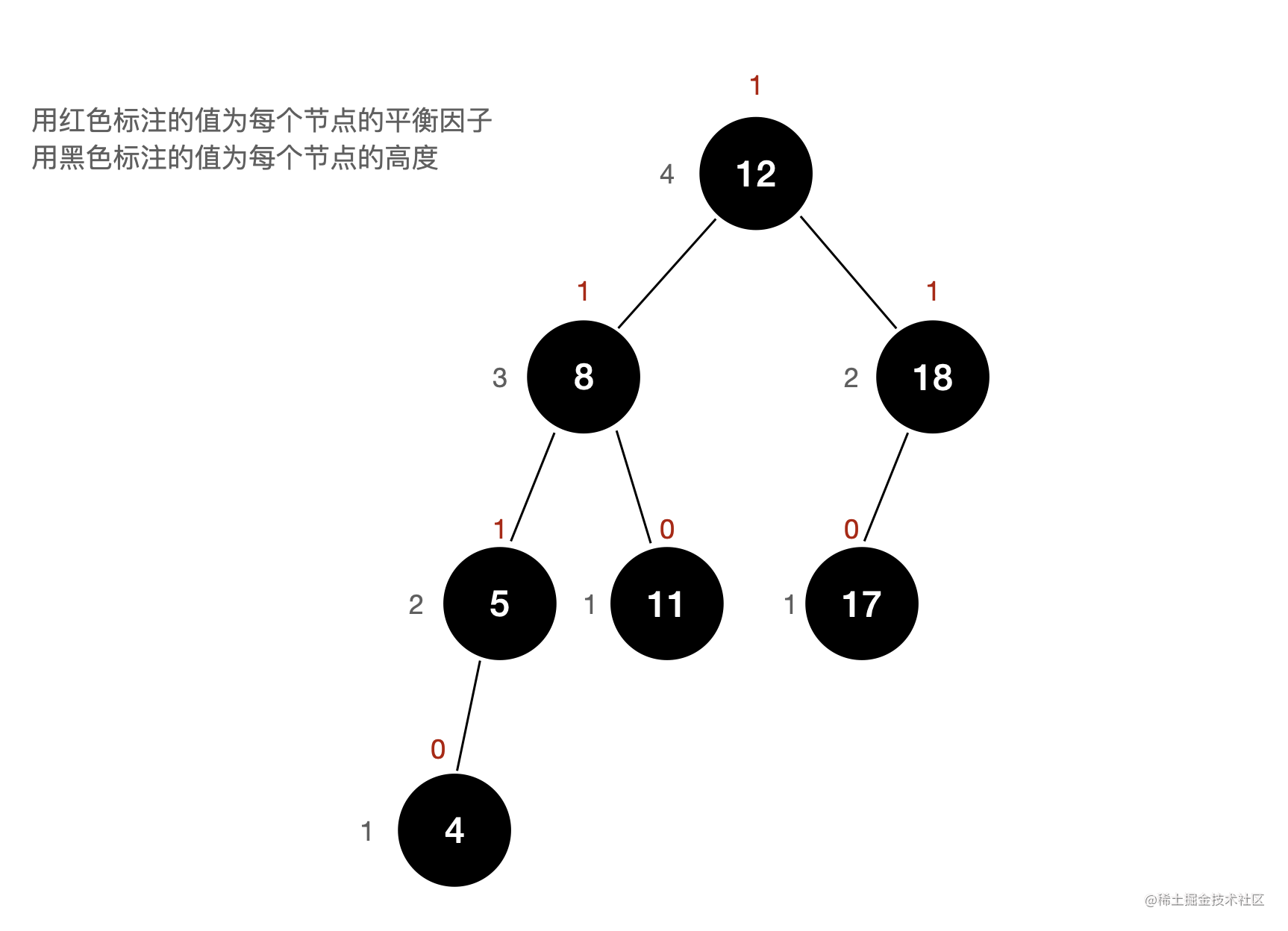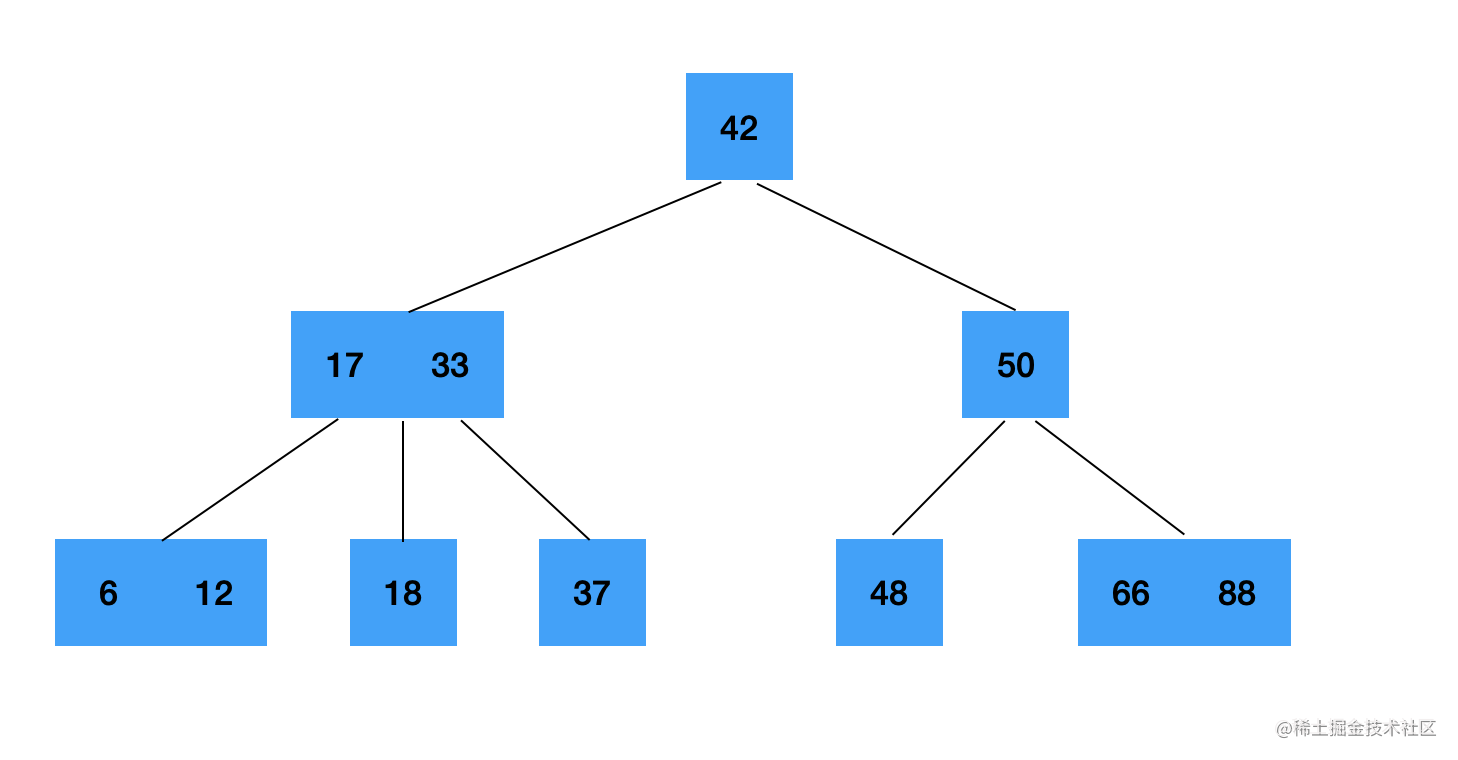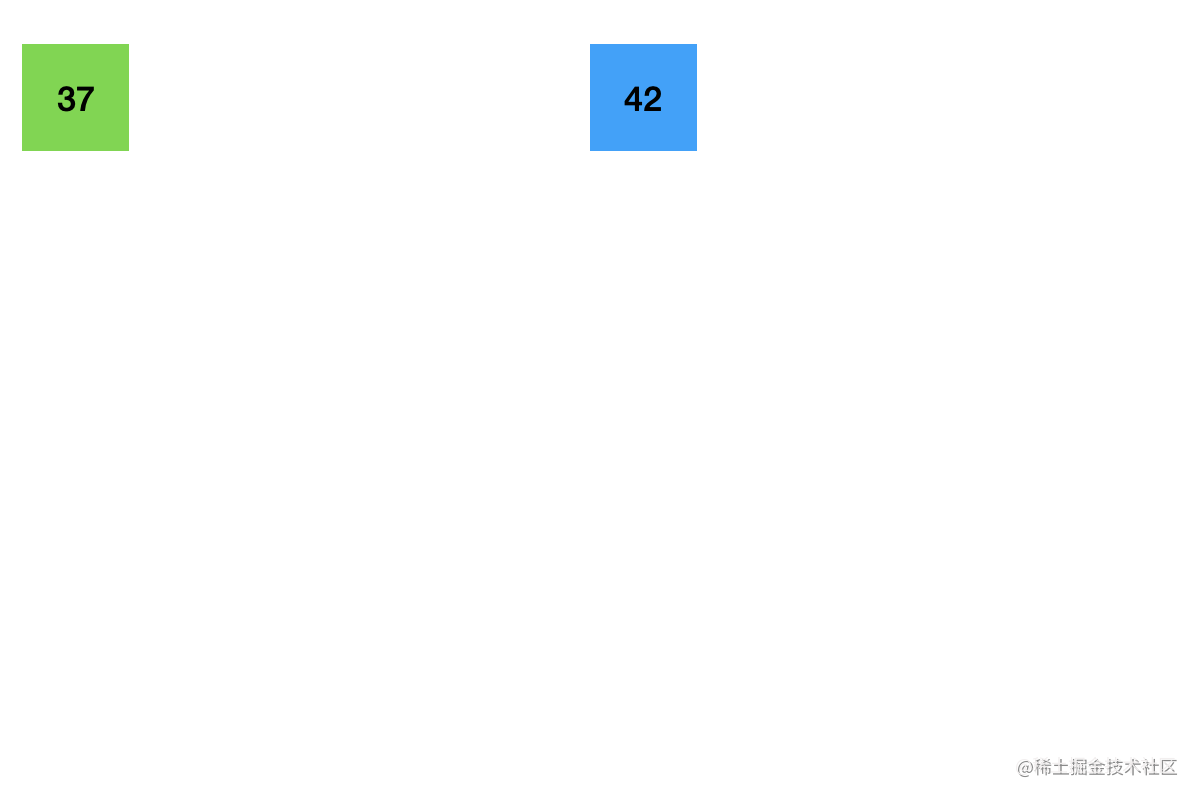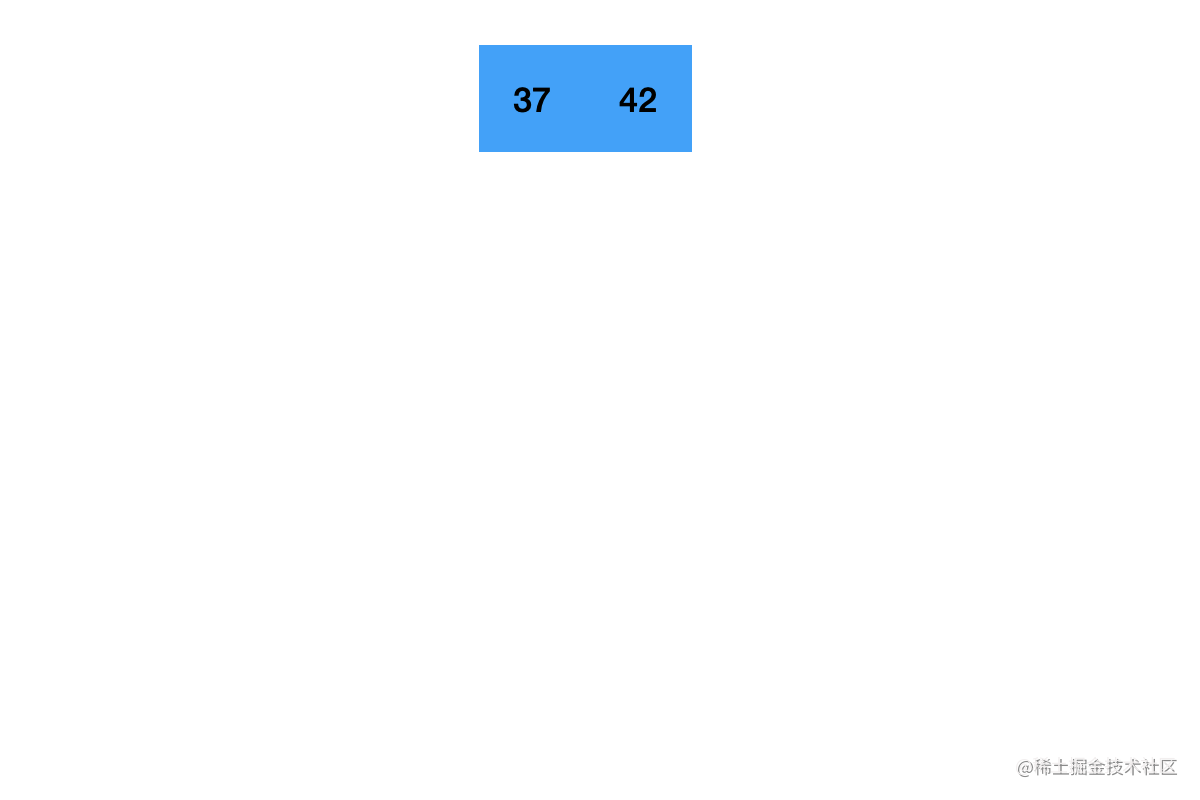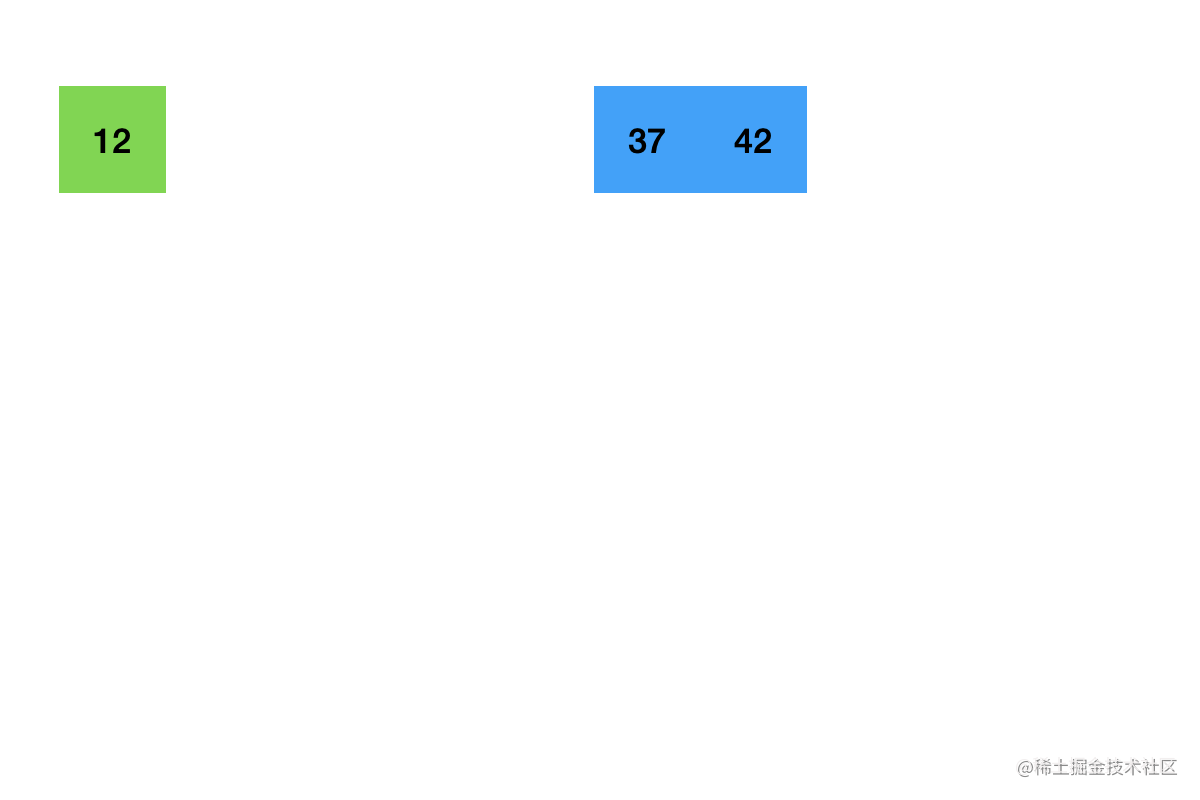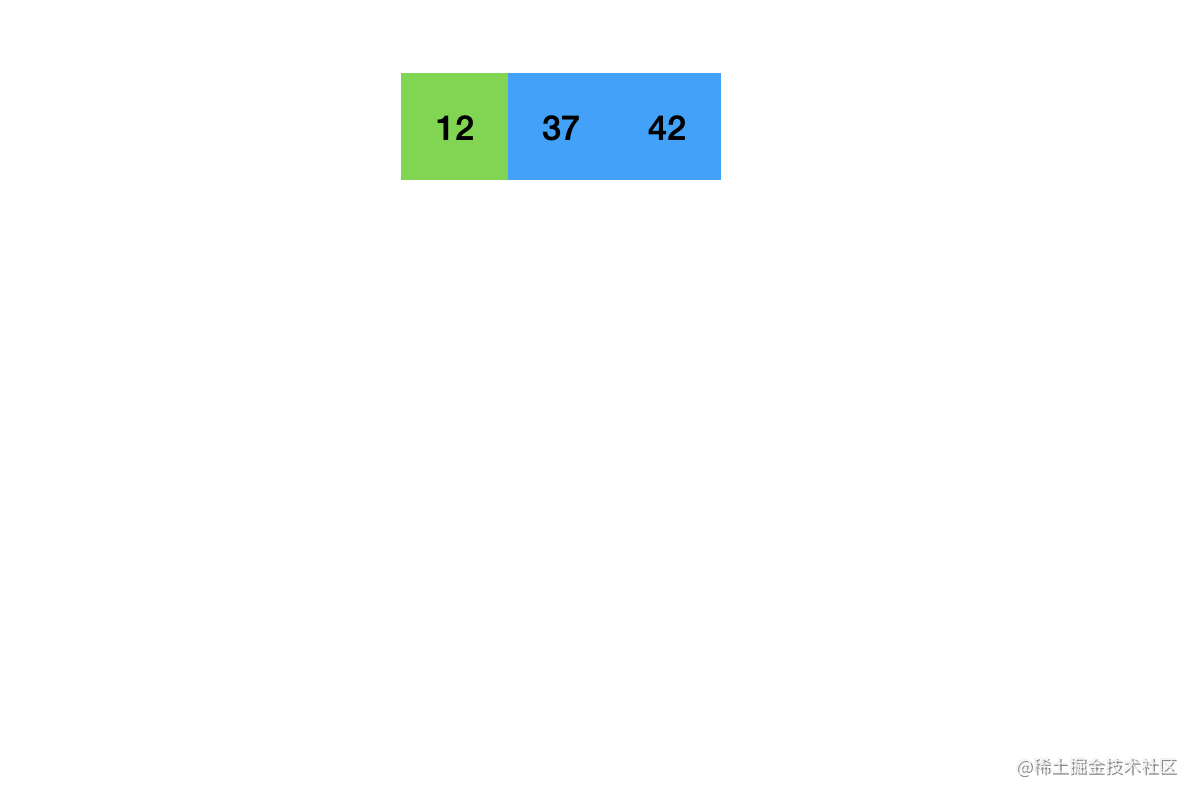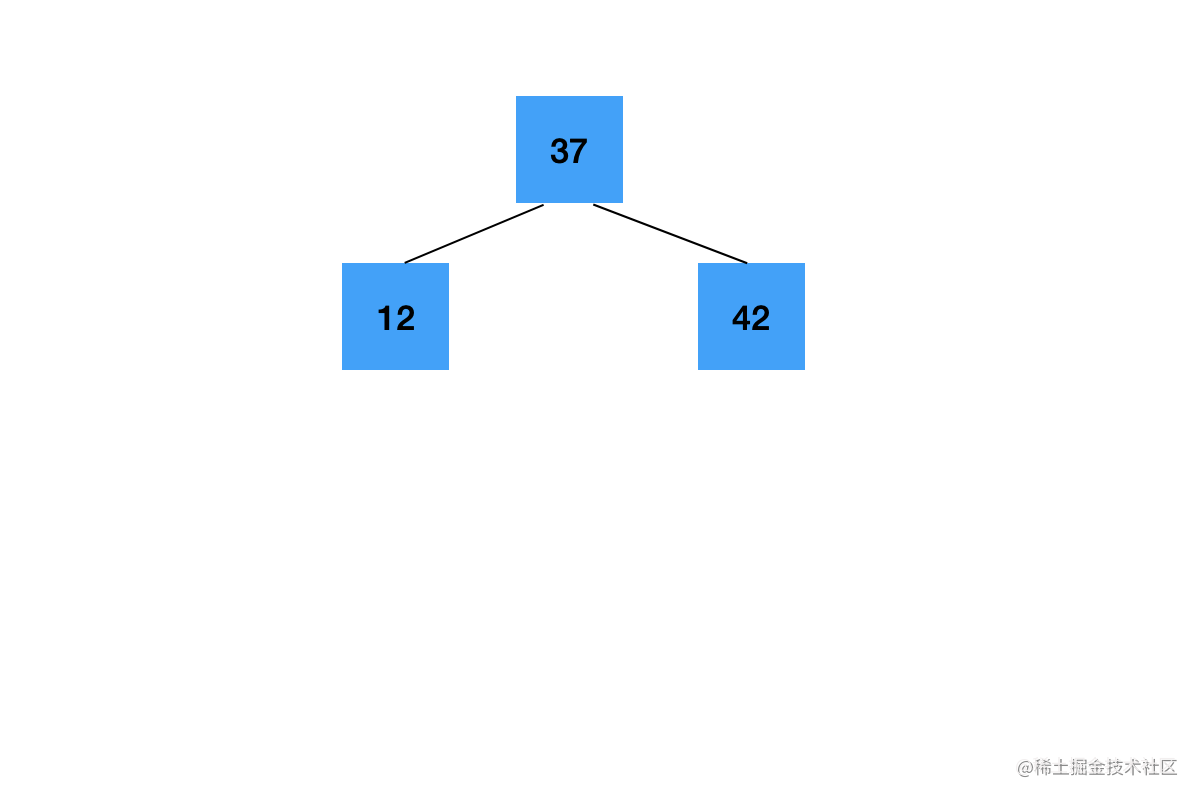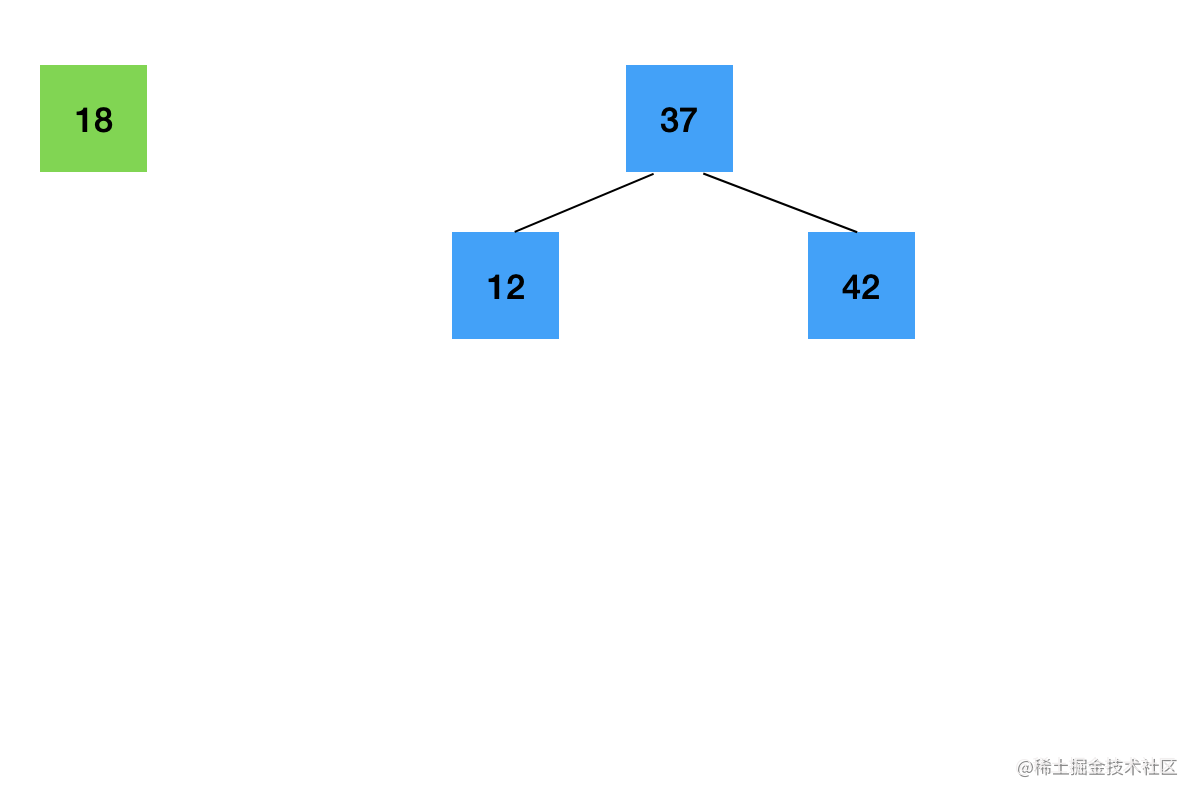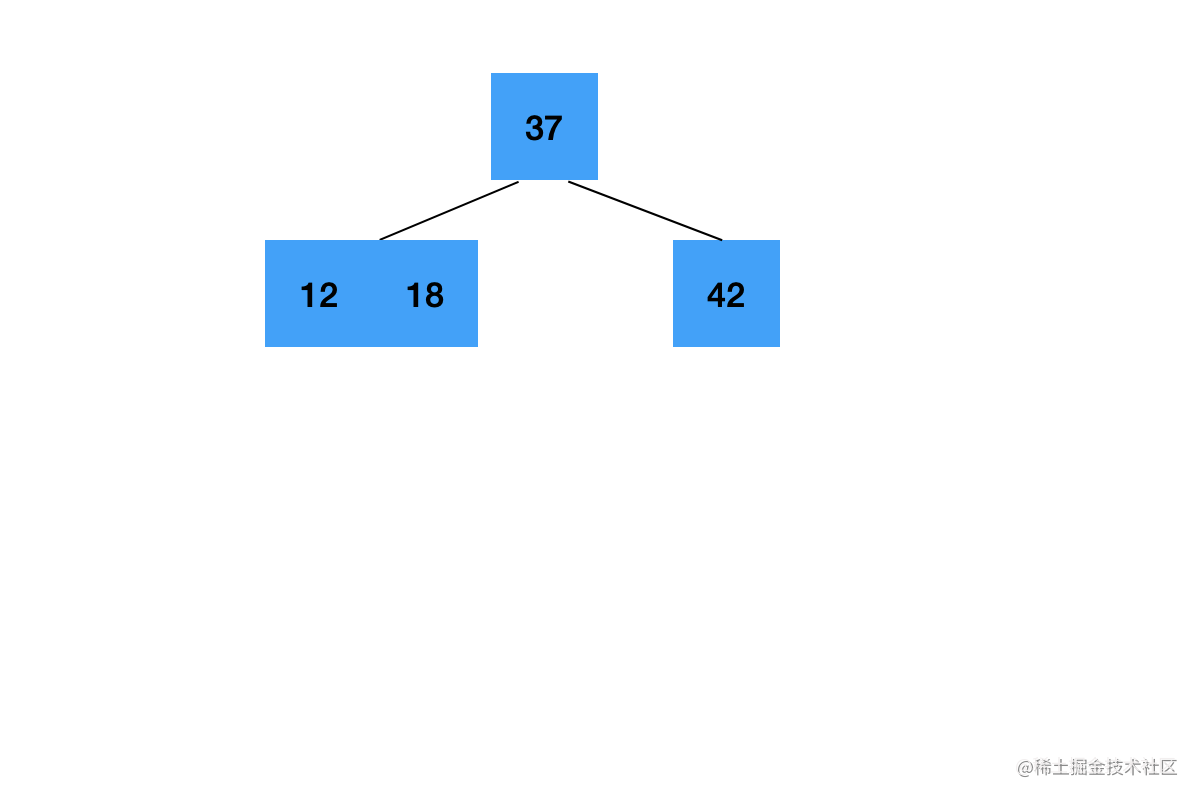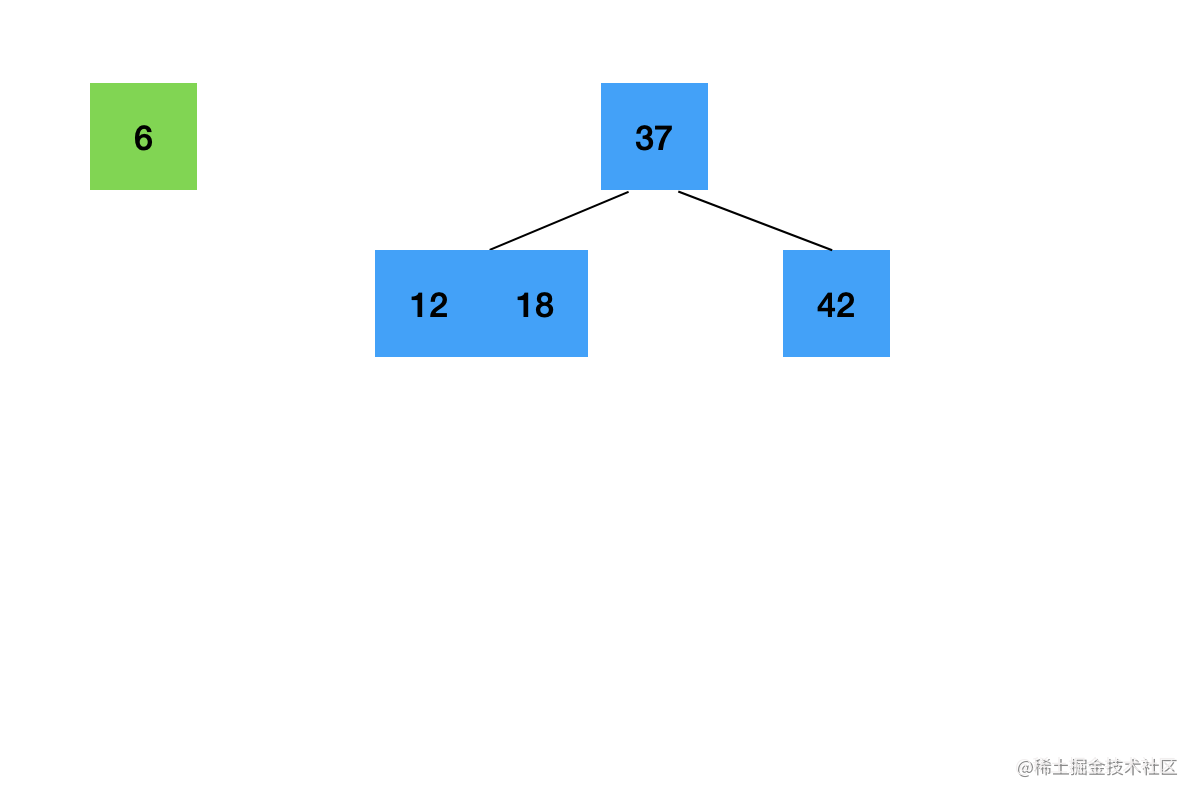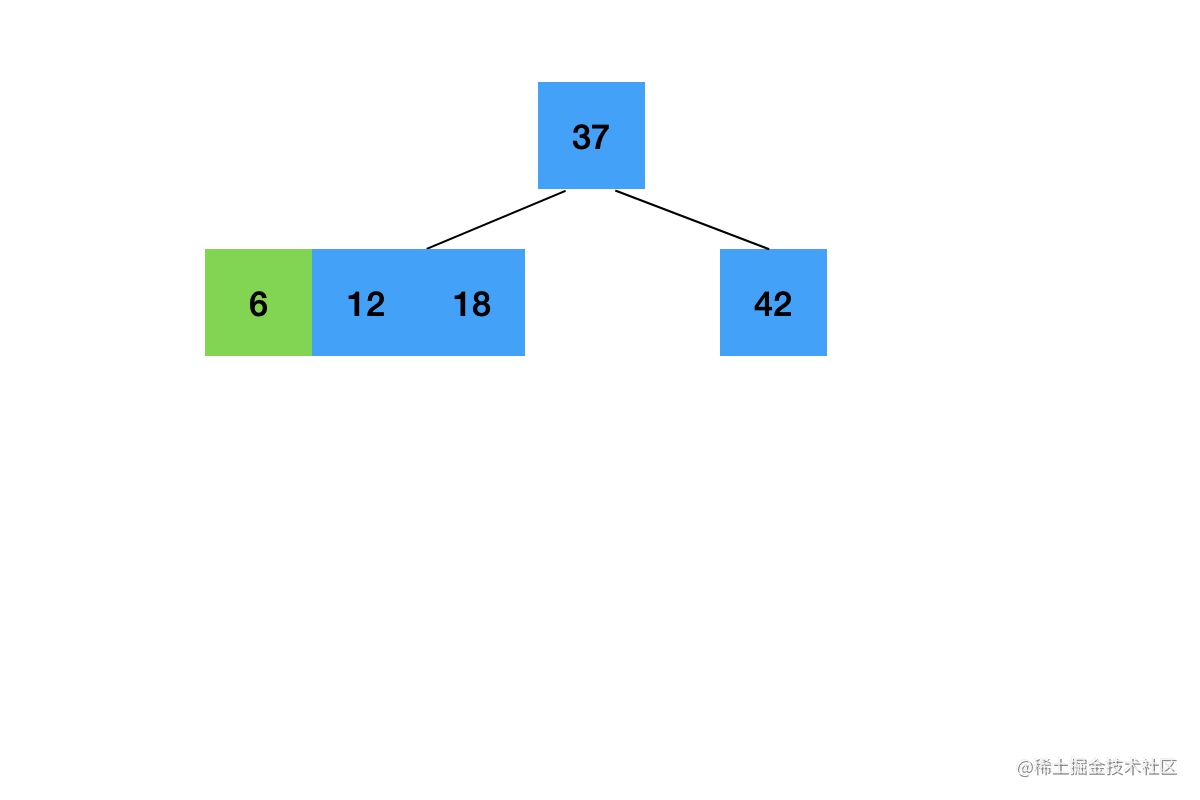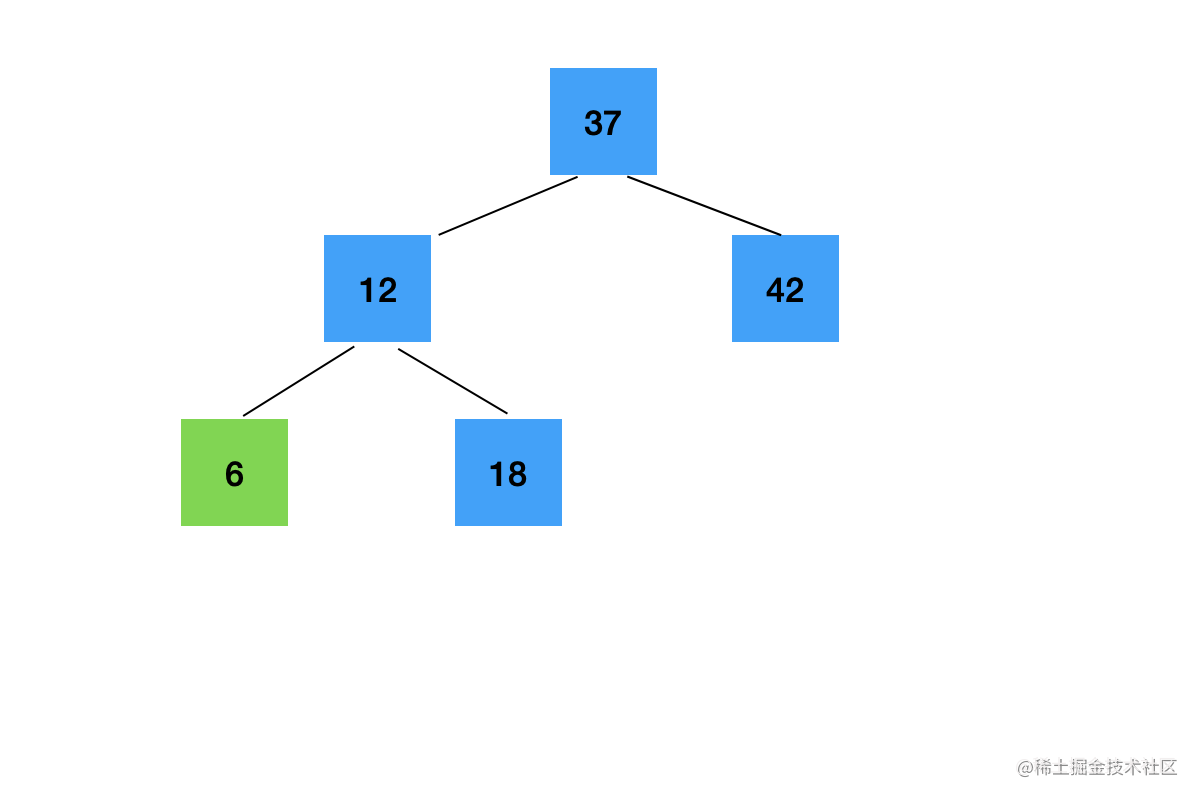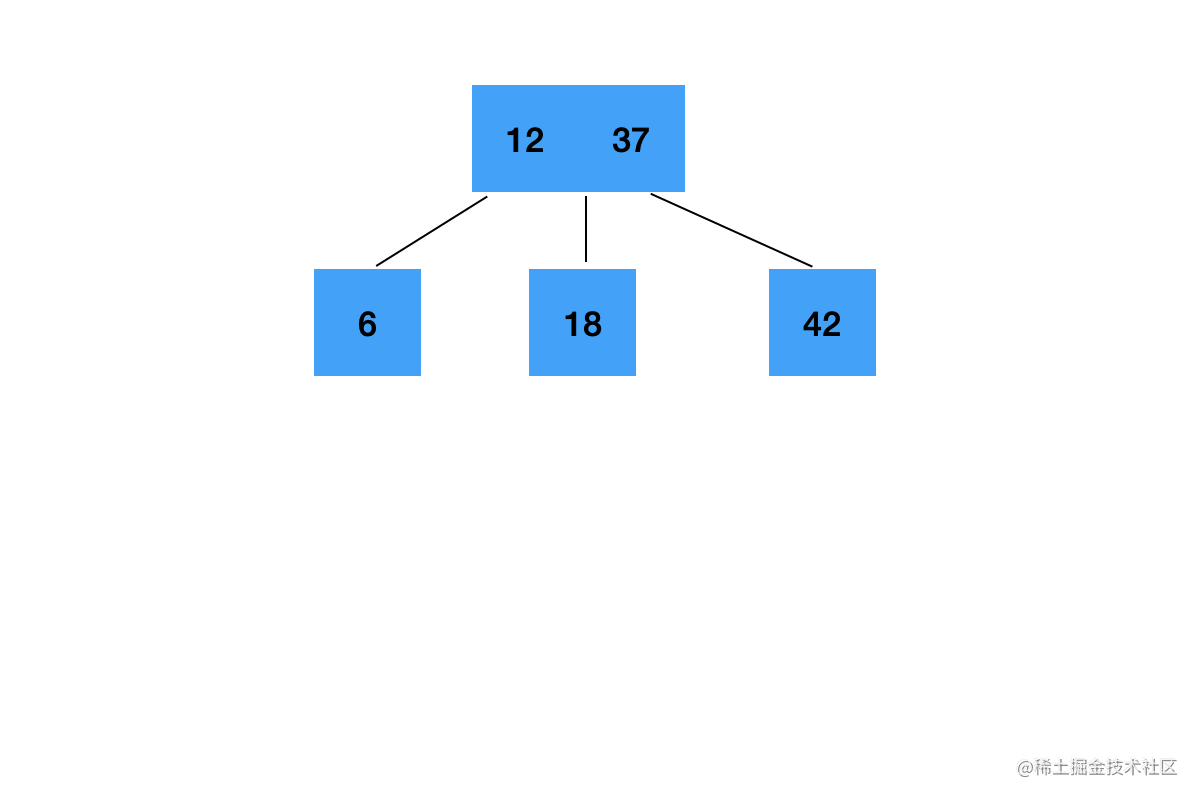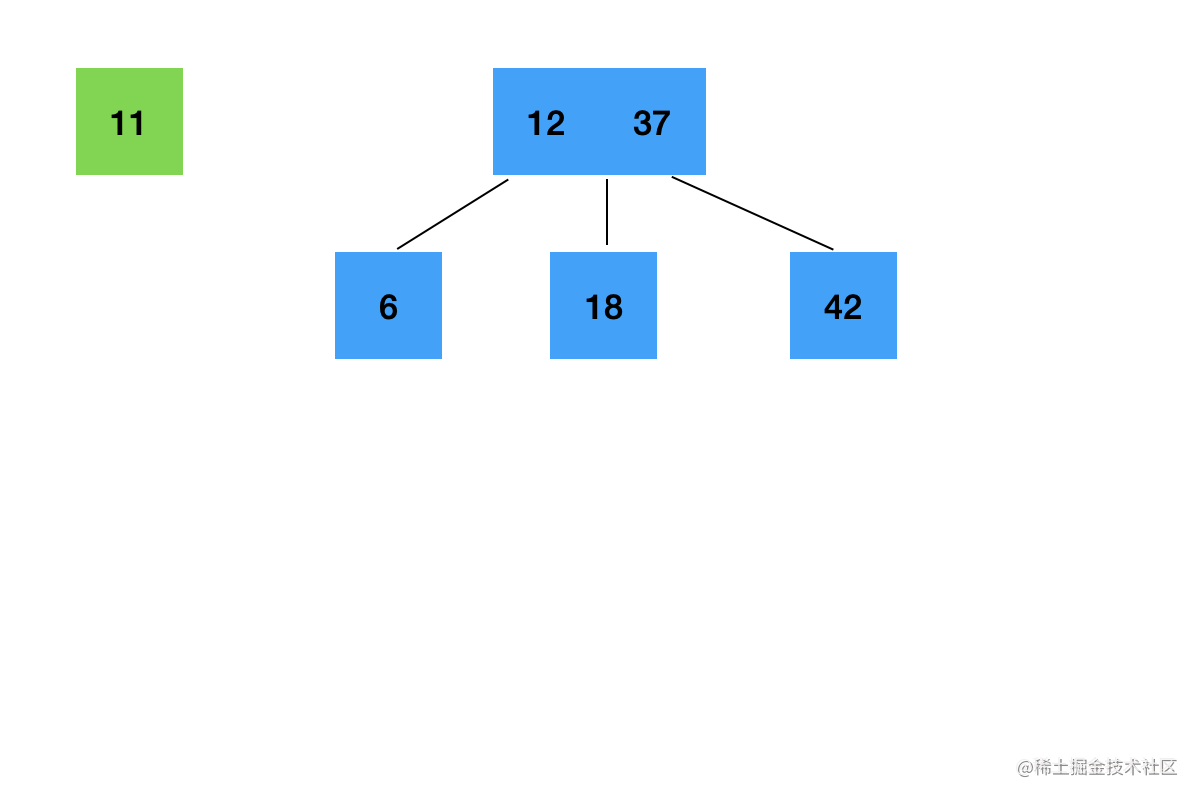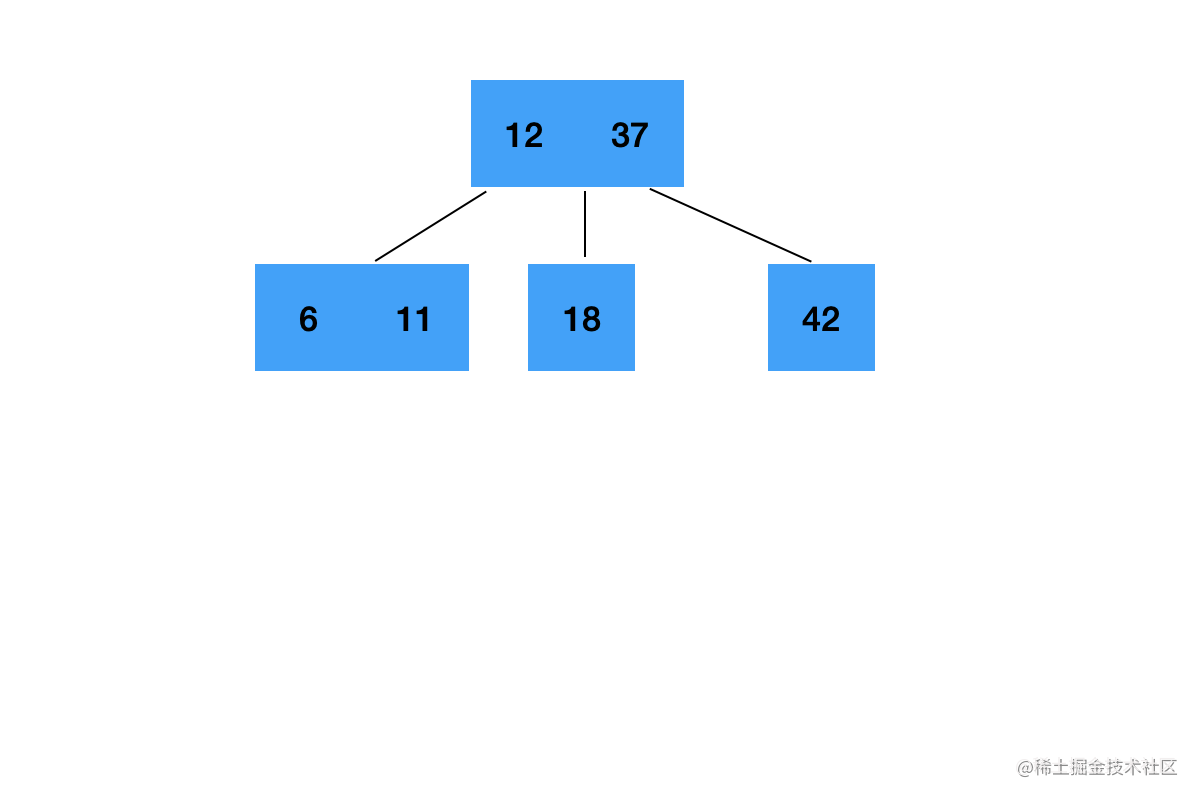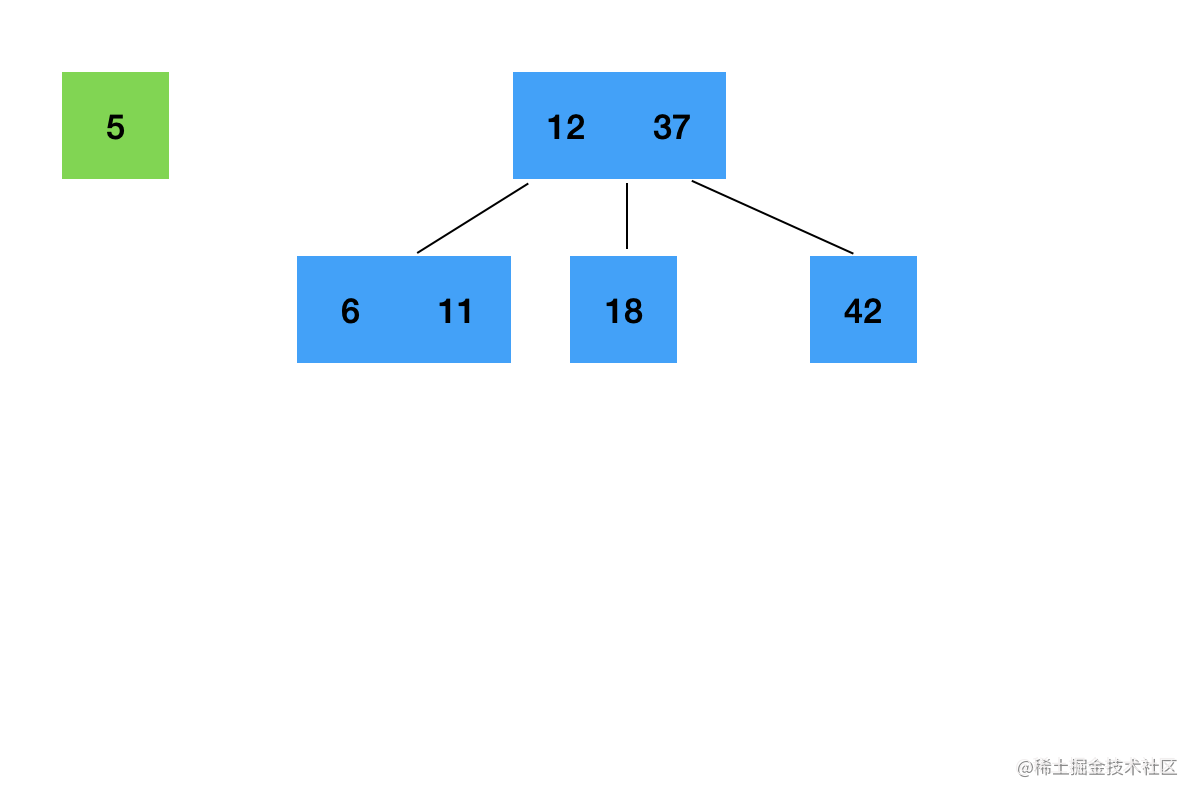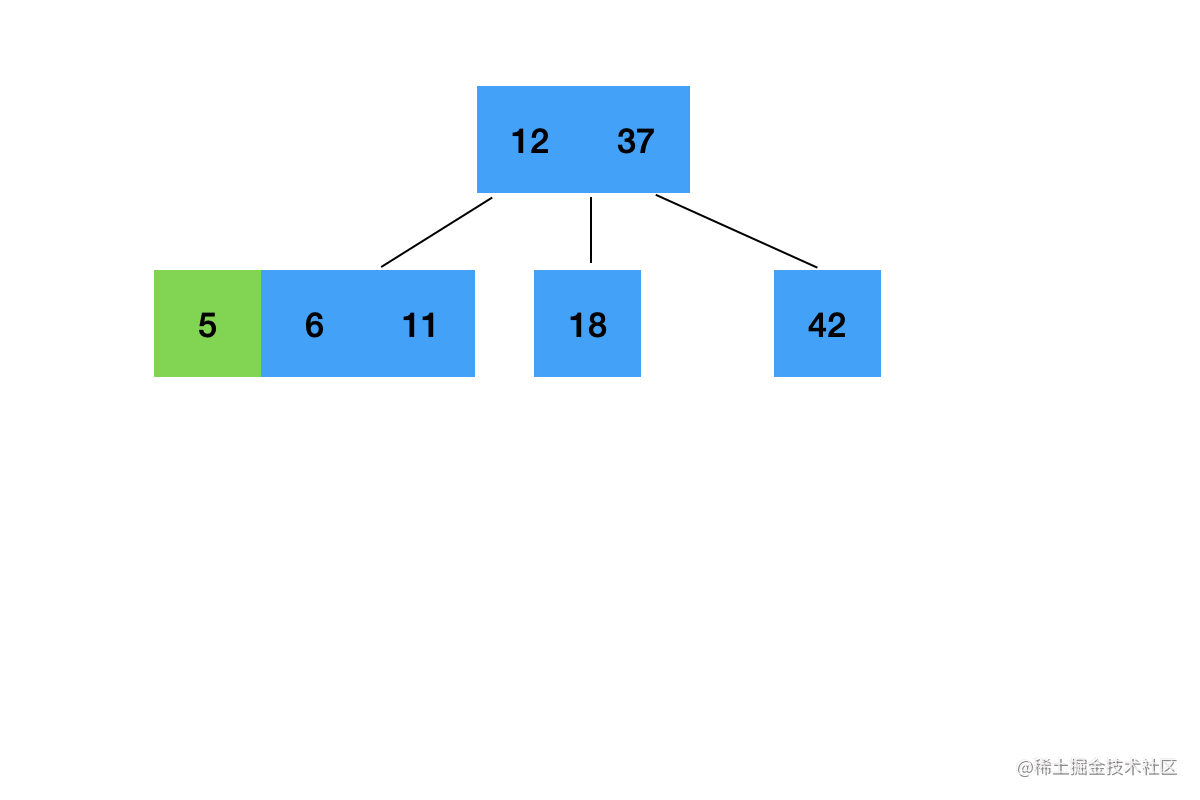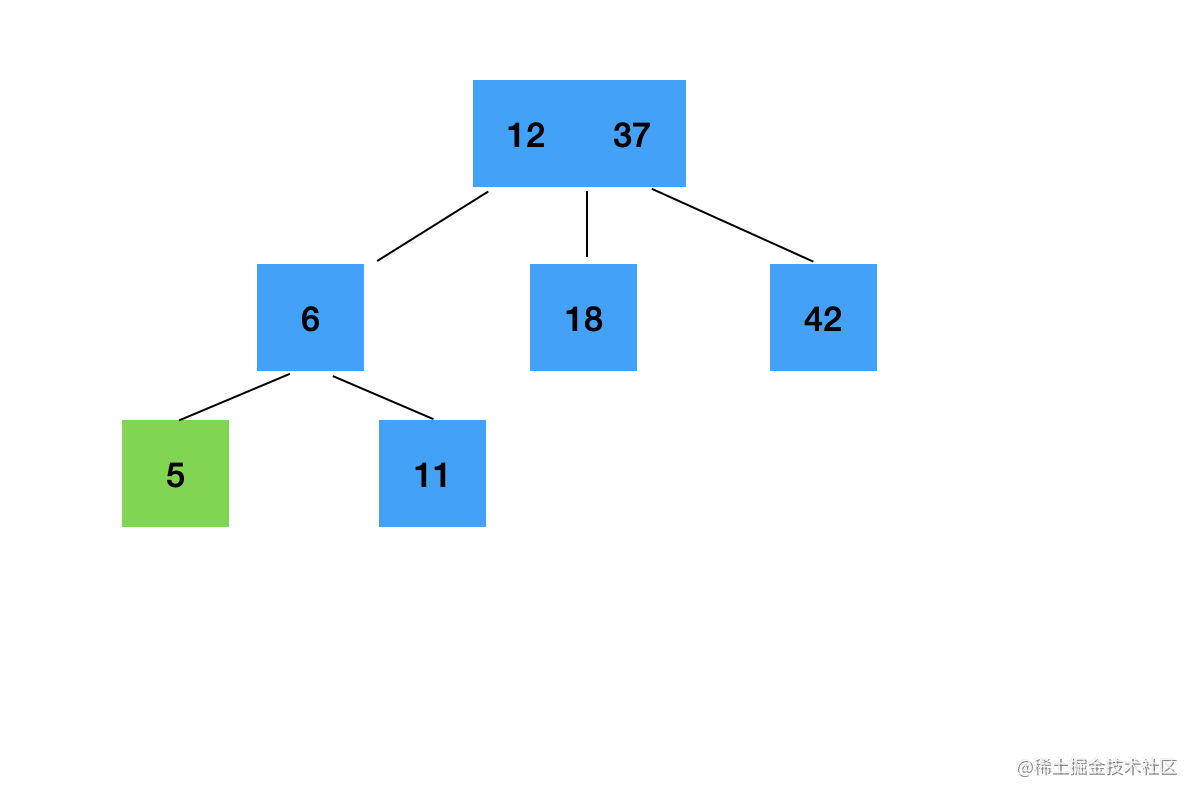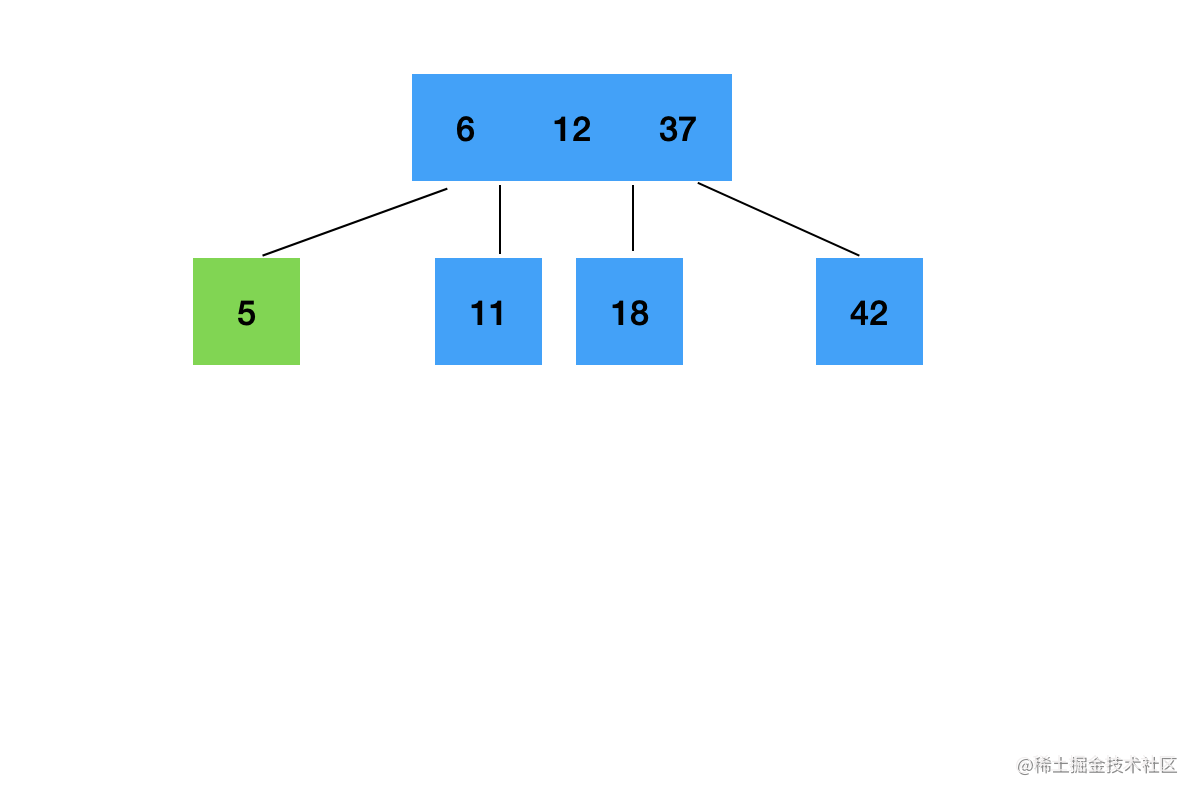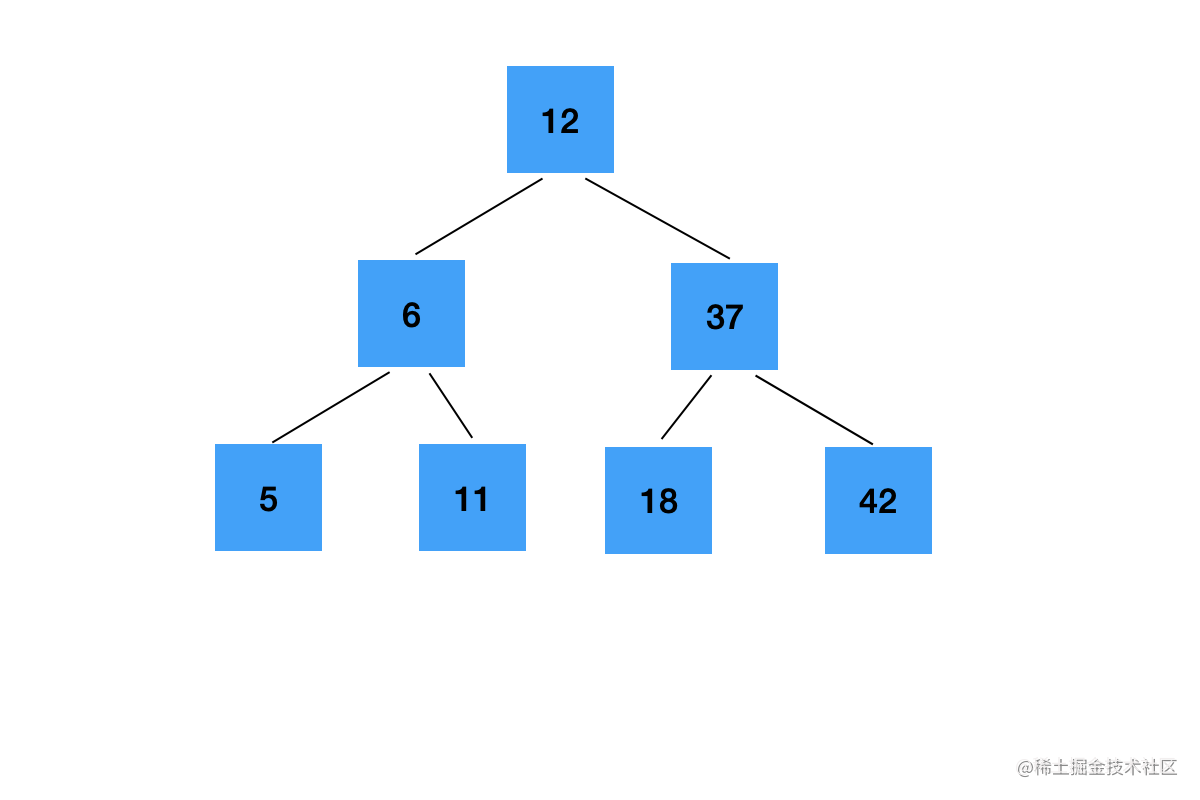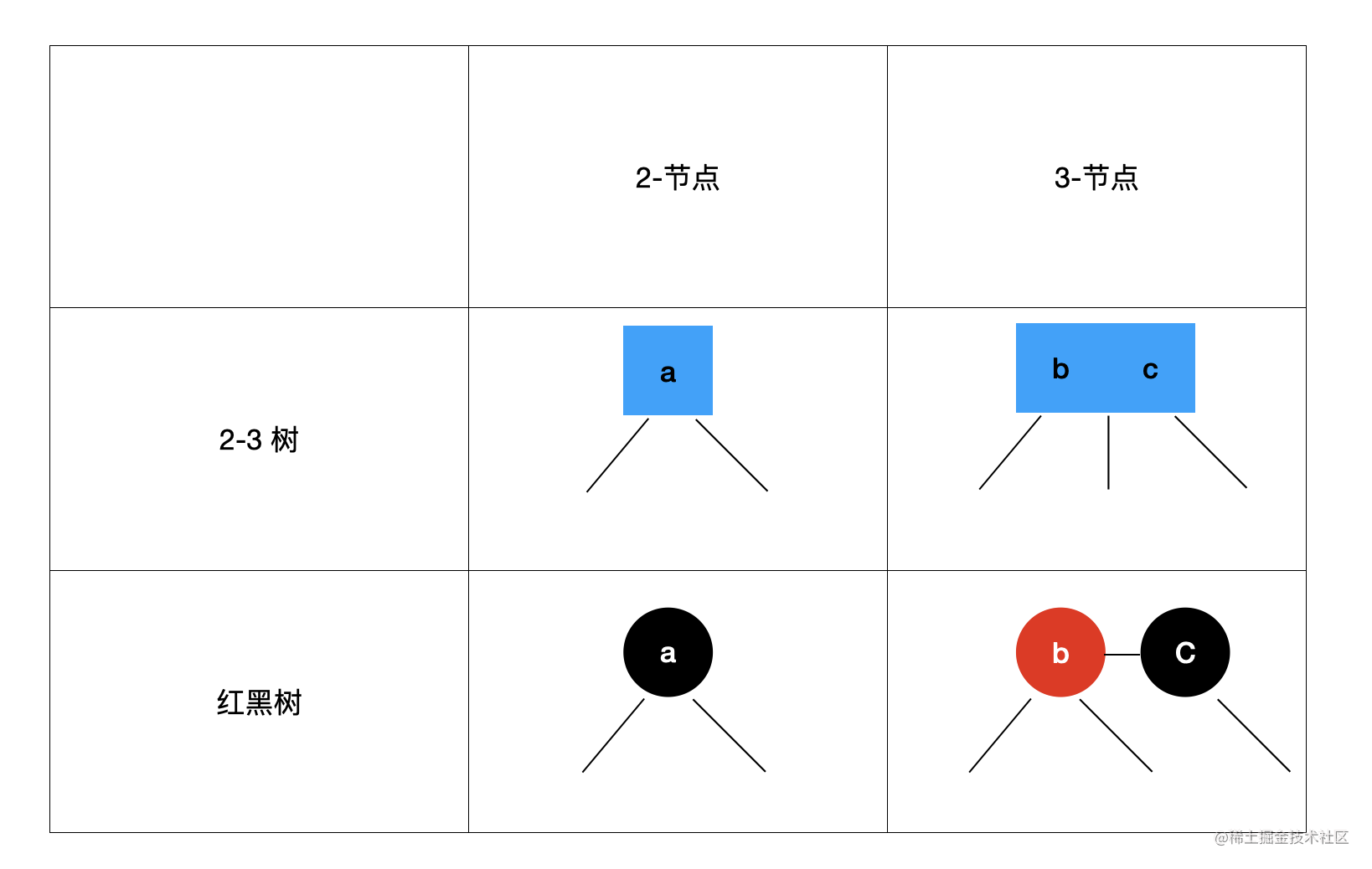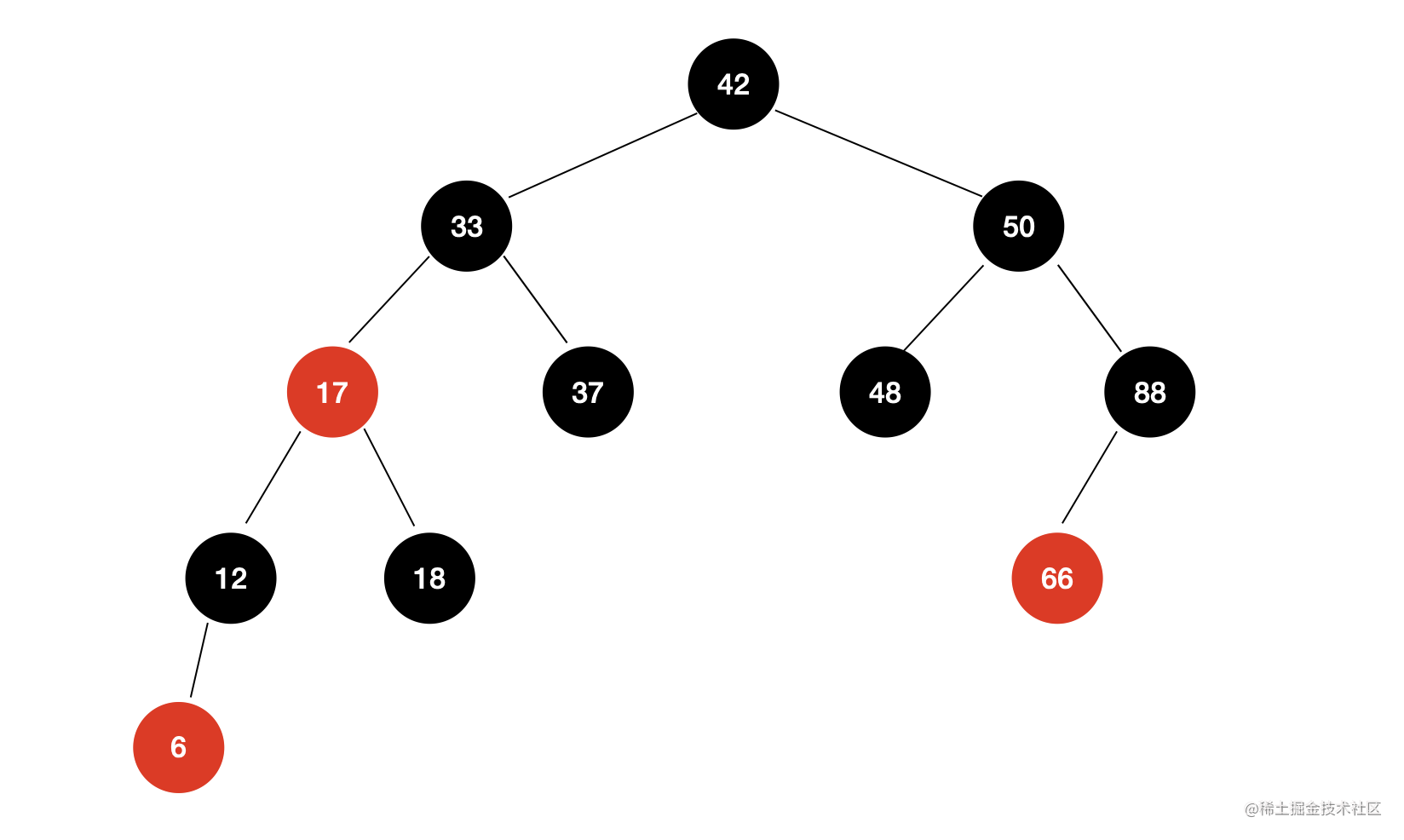## 红黑树的实现

### 红黑树的基本性质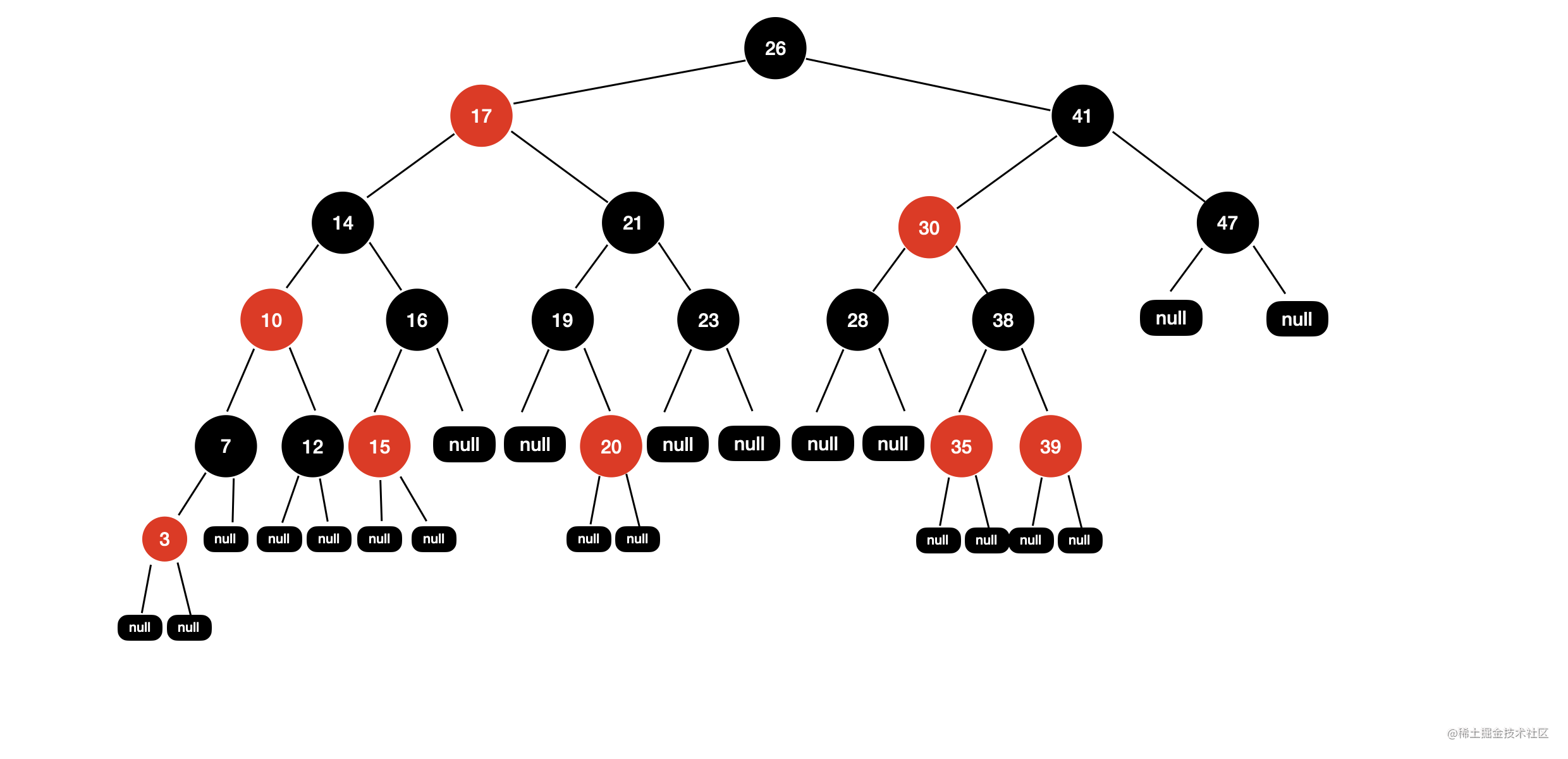1. 每个节点或者为红色，或者为黑色

2. 根节点的颜色一定是黑色

3. 每一个叶子节点（最后的空节点）一定是黑色的

4. 如果一个节点是红色的，那么它的孩子节点都是黑色的

5. 从任意一个节点到叶子节点，经过的黑色节点的数量一样多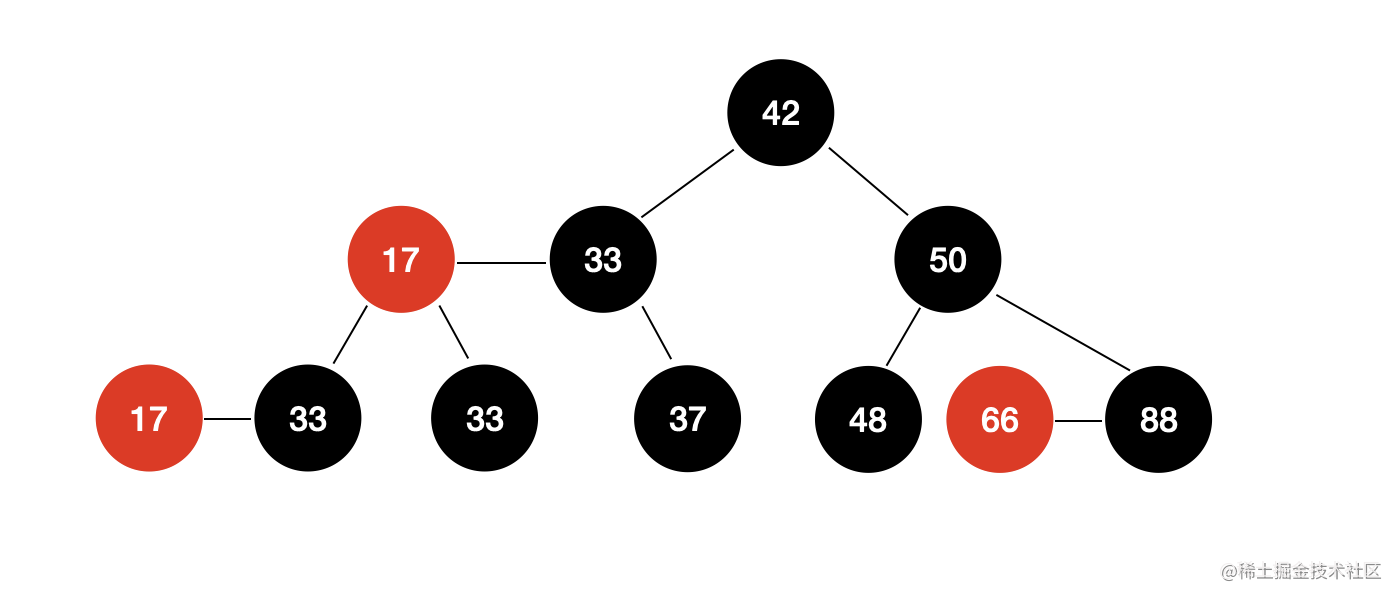### 向红黑树中添加元素的代码实现

public class Node<E> {

public E e;
public Node left;
public Node right;
public boolean color;

public Node(E e) {
this.e = e;
left = null;
right = null;
color = true; // true 表示红色，false 表示黑色
}
}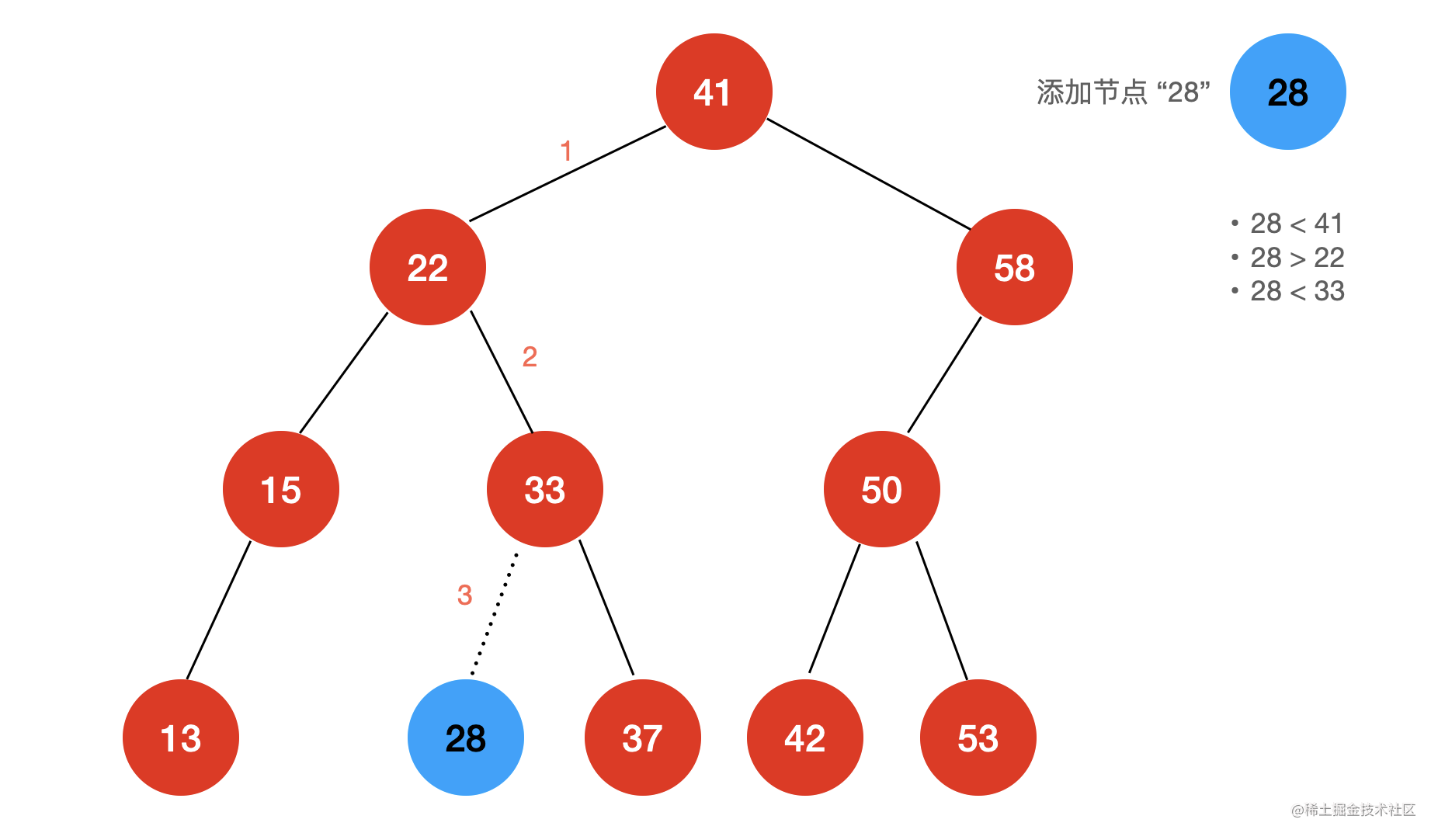Java 代码：

/**
* @param e 向二分搜索树中添加新的元素
*/
}

/**
* @param e    向二分搜索树中新插入的节点
* @param node 当前比较的节点
* @return 返回二分搜索树的根节点
*/
private Node add(E e, Node node) {
if (node == null) {
size++;
return new Node(e);
}
if (e.compareTo((E) node.e) < 0) {
} else if (e.compareTo((E) node.e) > 0) {
}
return node;
}

public void add(E e) {
root.color = BLACK; // false
}

• 当前插入的节点与 2-节点进行融合
• 当前插入的节点与 3-节点进行融合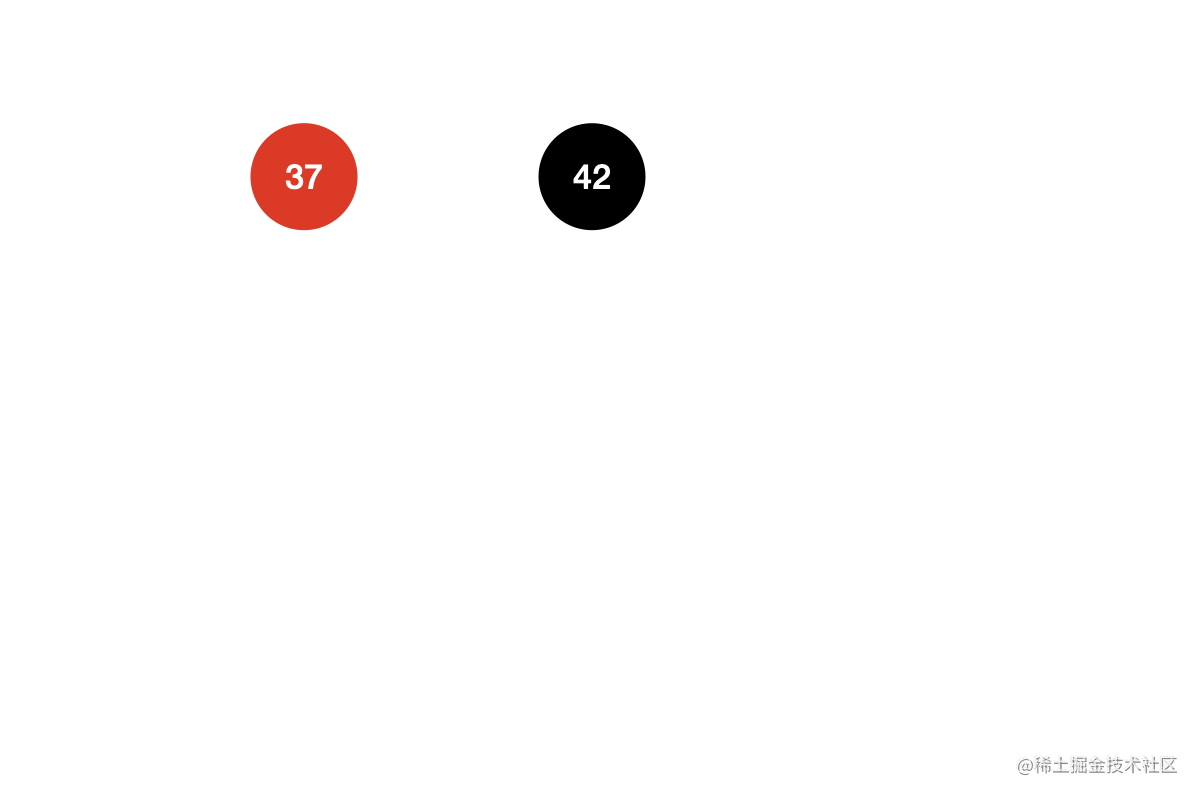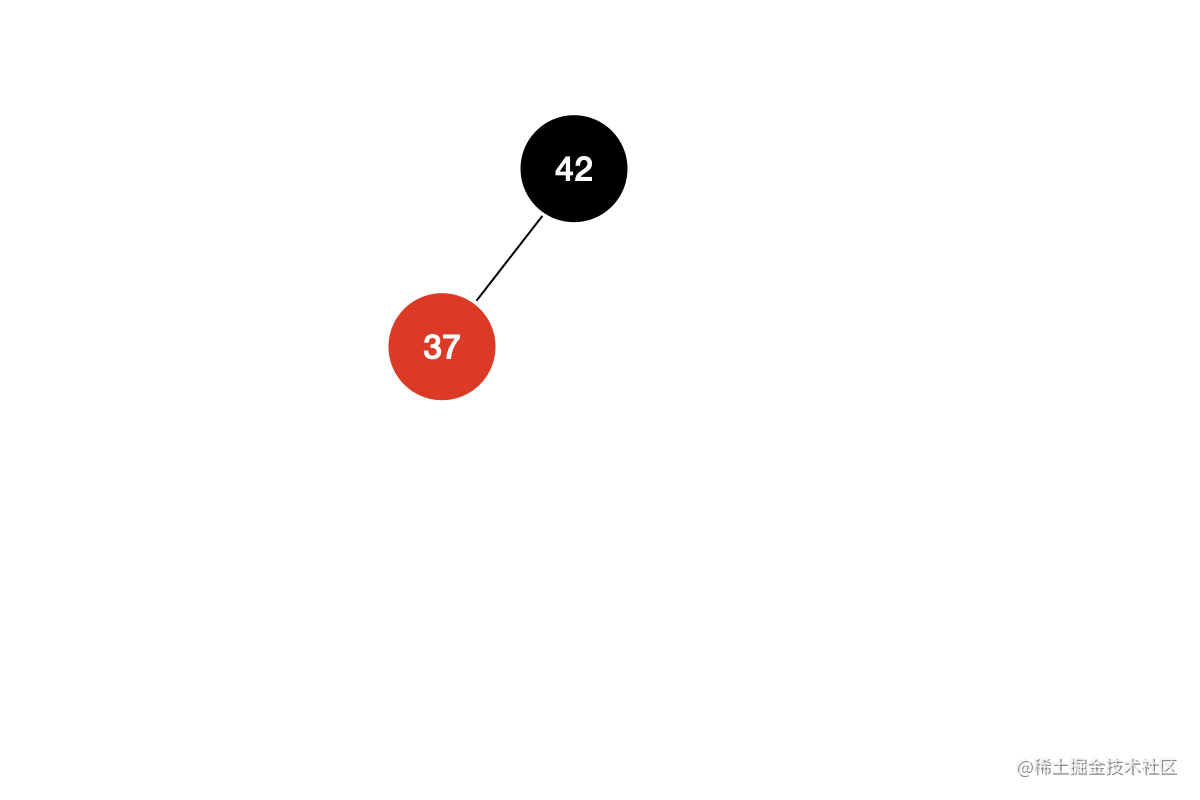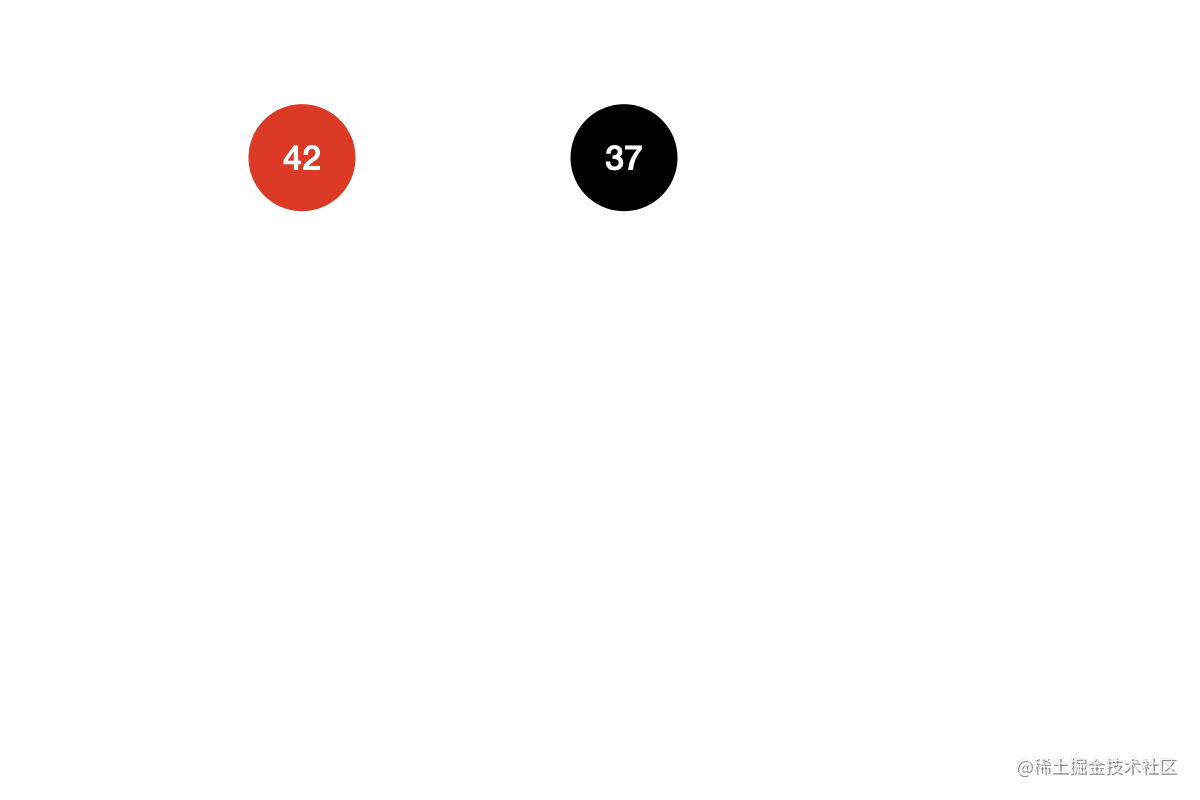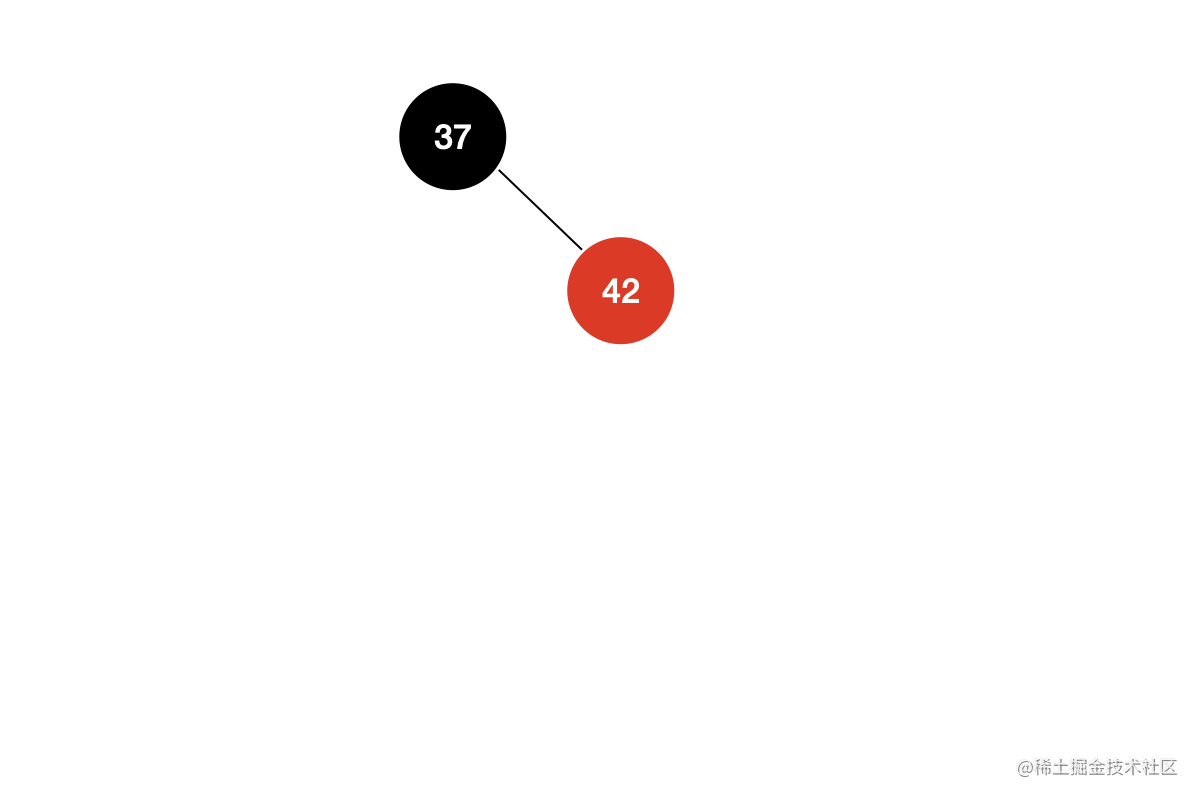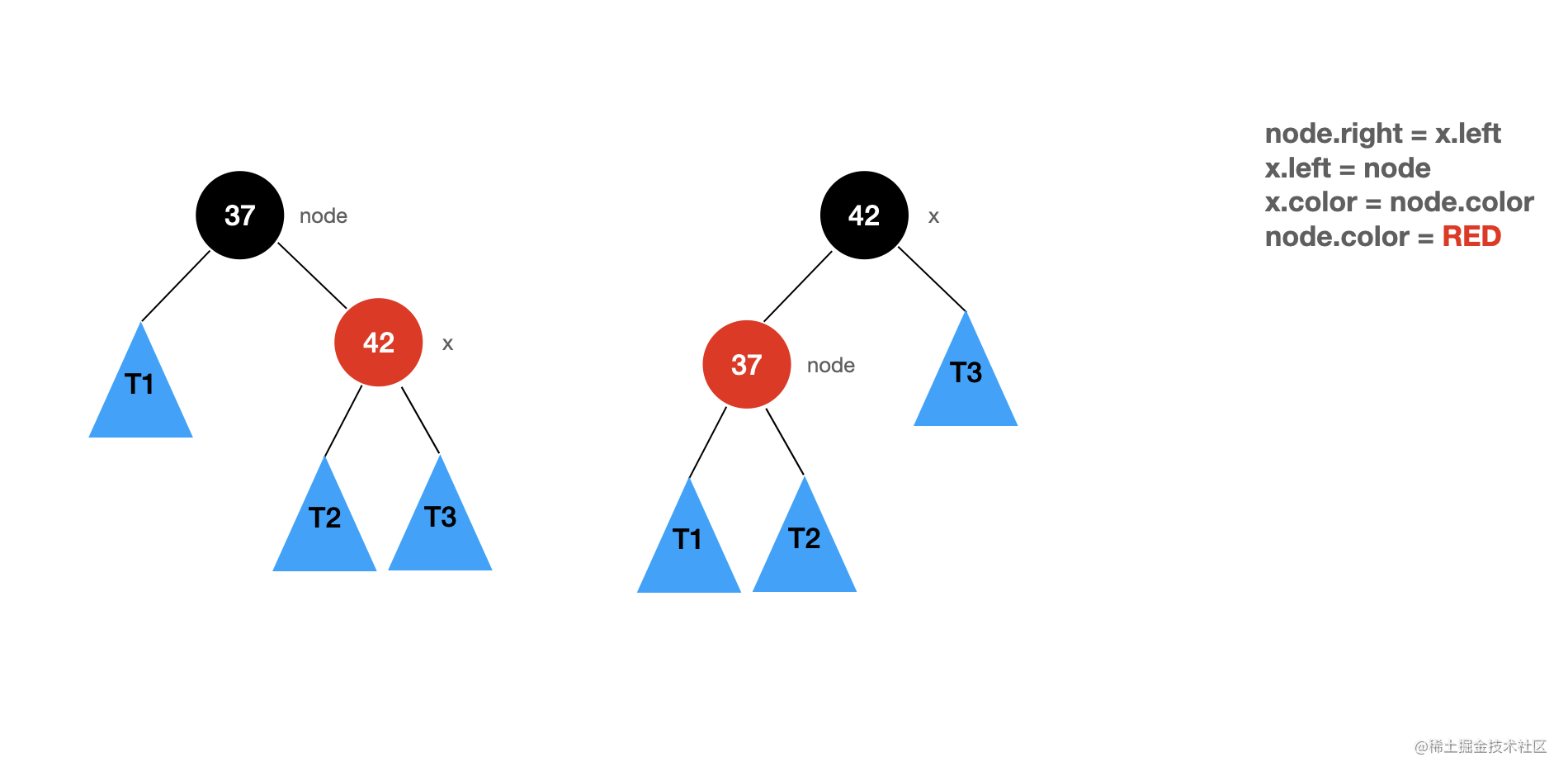/**
* 左旋转:
*
*        node                      x
*       /   \                    /  \
*     T1     x    =========>   node T3
*          /  \               /   \
*         T2  T3             T1   T2
*
*/
private Node leftRotate(Node node){
Node x = node.right;

// 左旋转
node.right = x.left;
x.left = node;

x.color = node.color;
node.color = RED;

return x;
}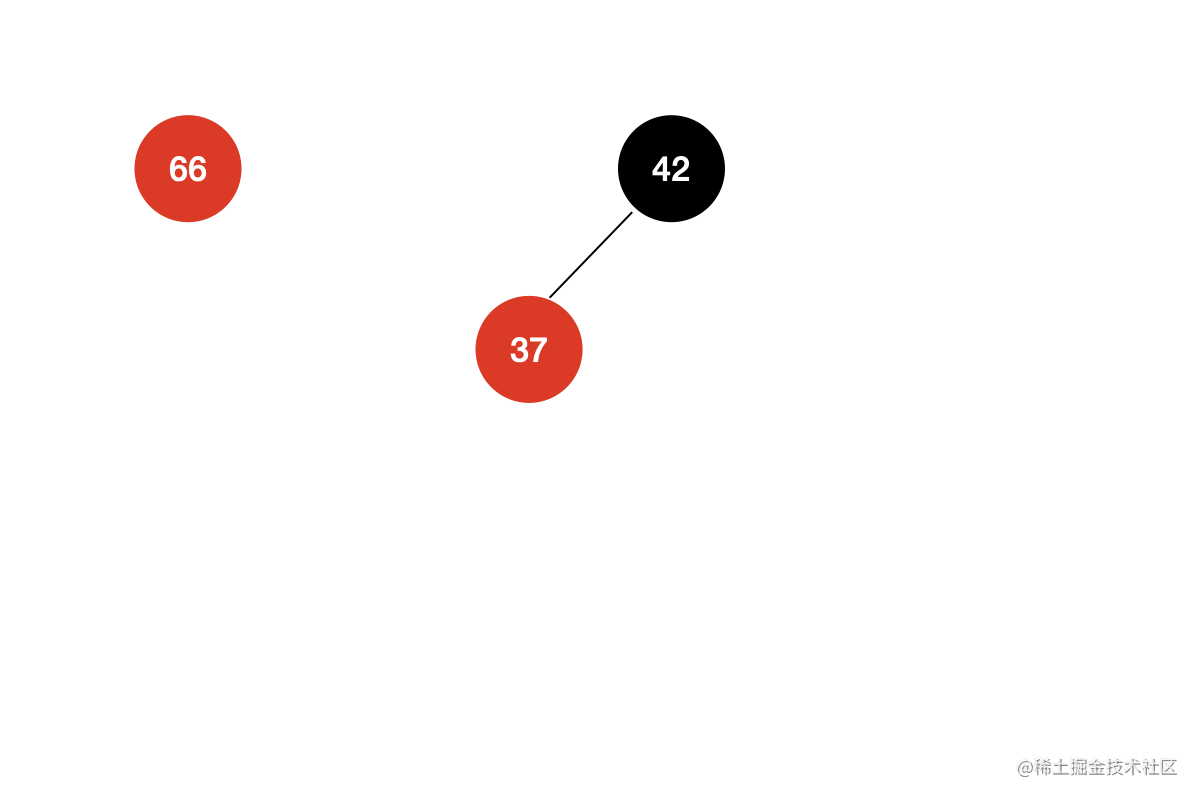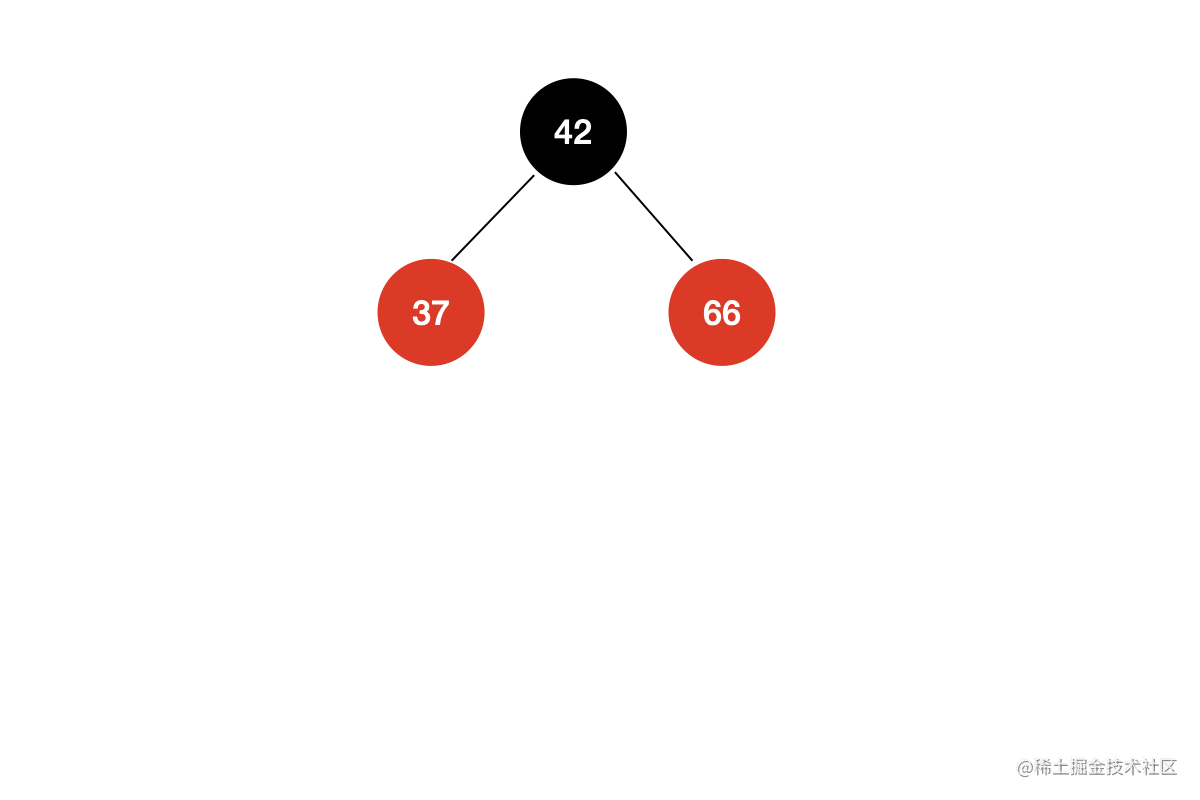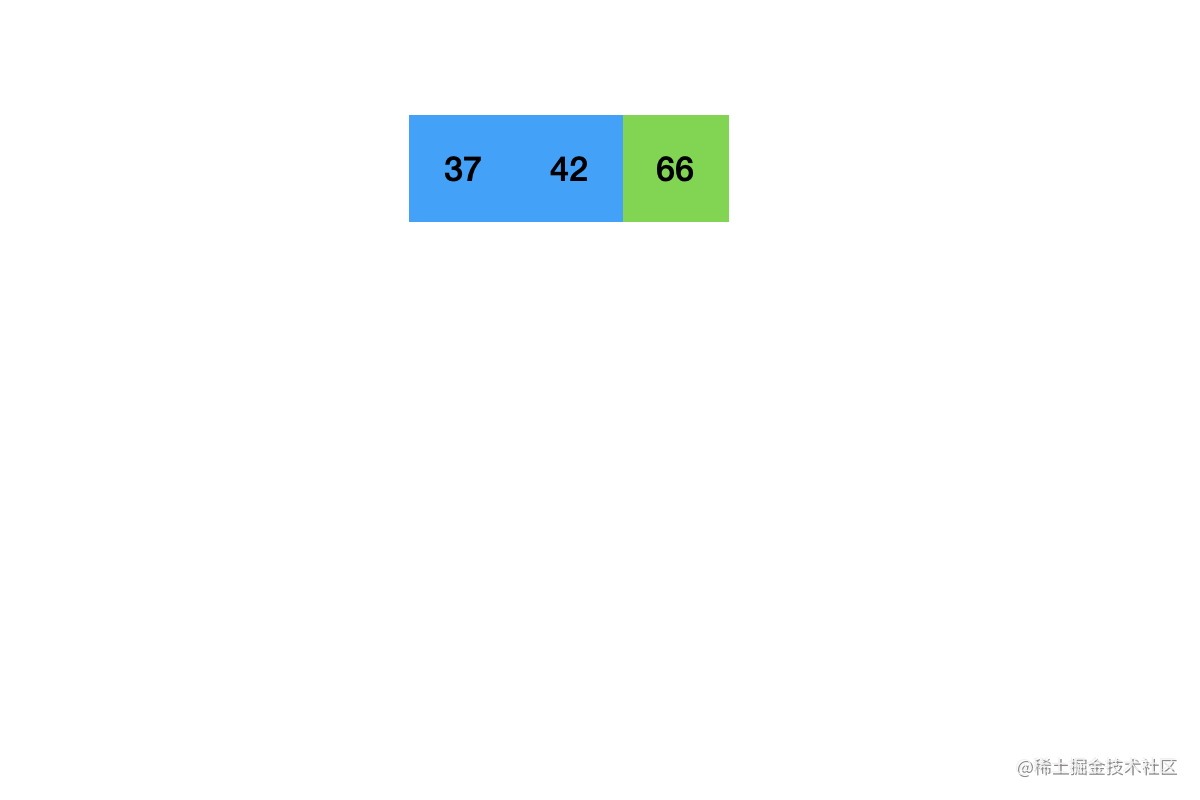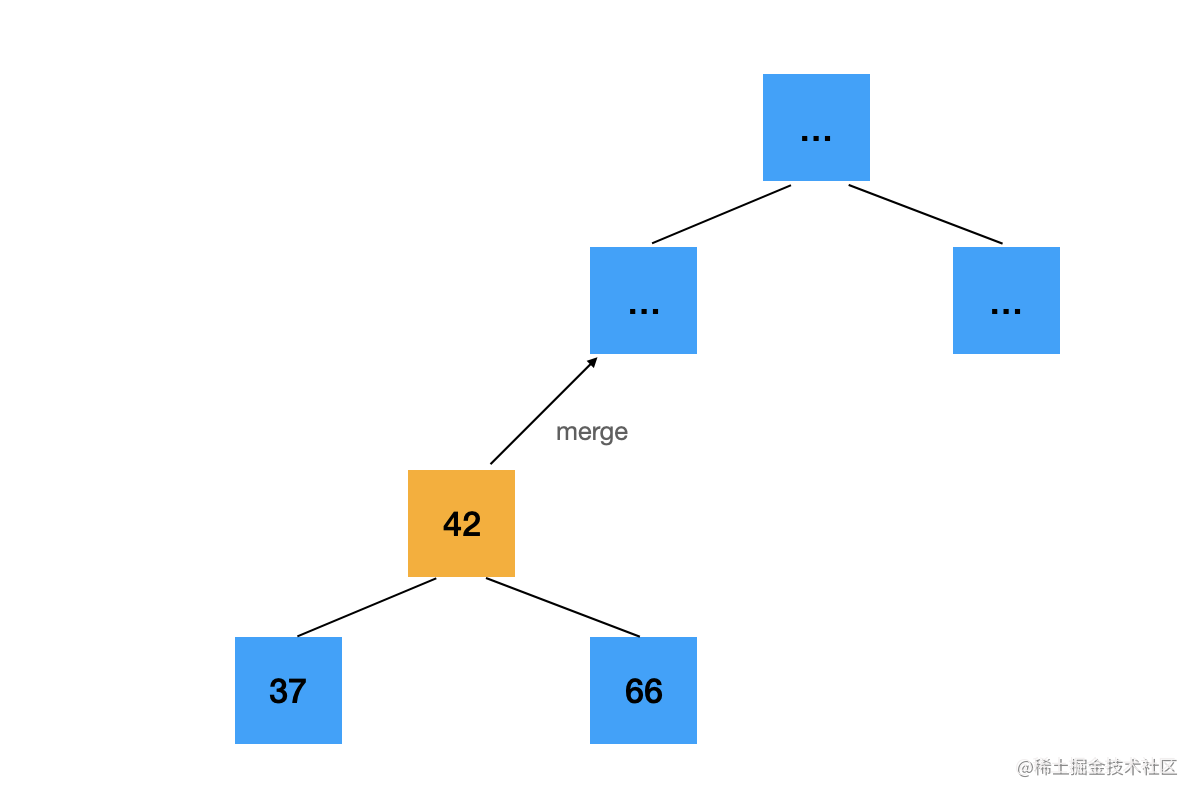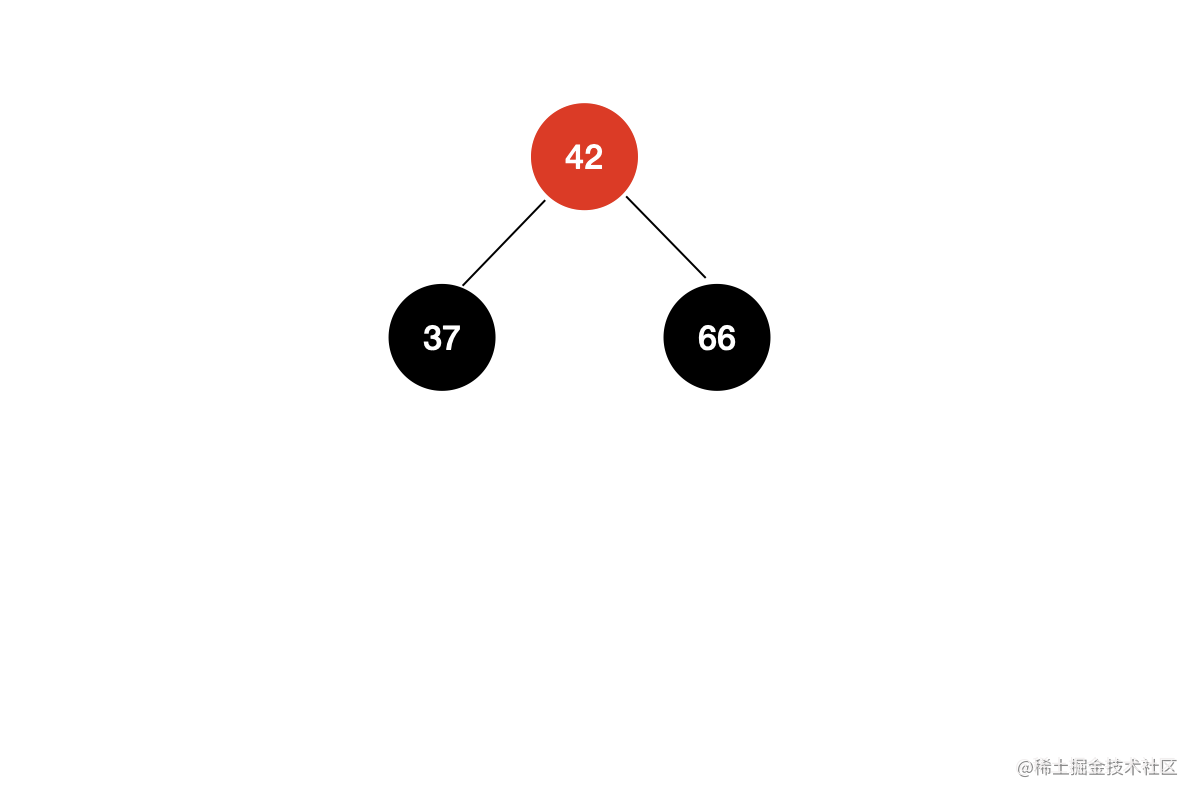// flipColors
private void flipColors(Node node){
node.color = RED;
node.left.color = BLACK;
node.right.color = BLACK;
}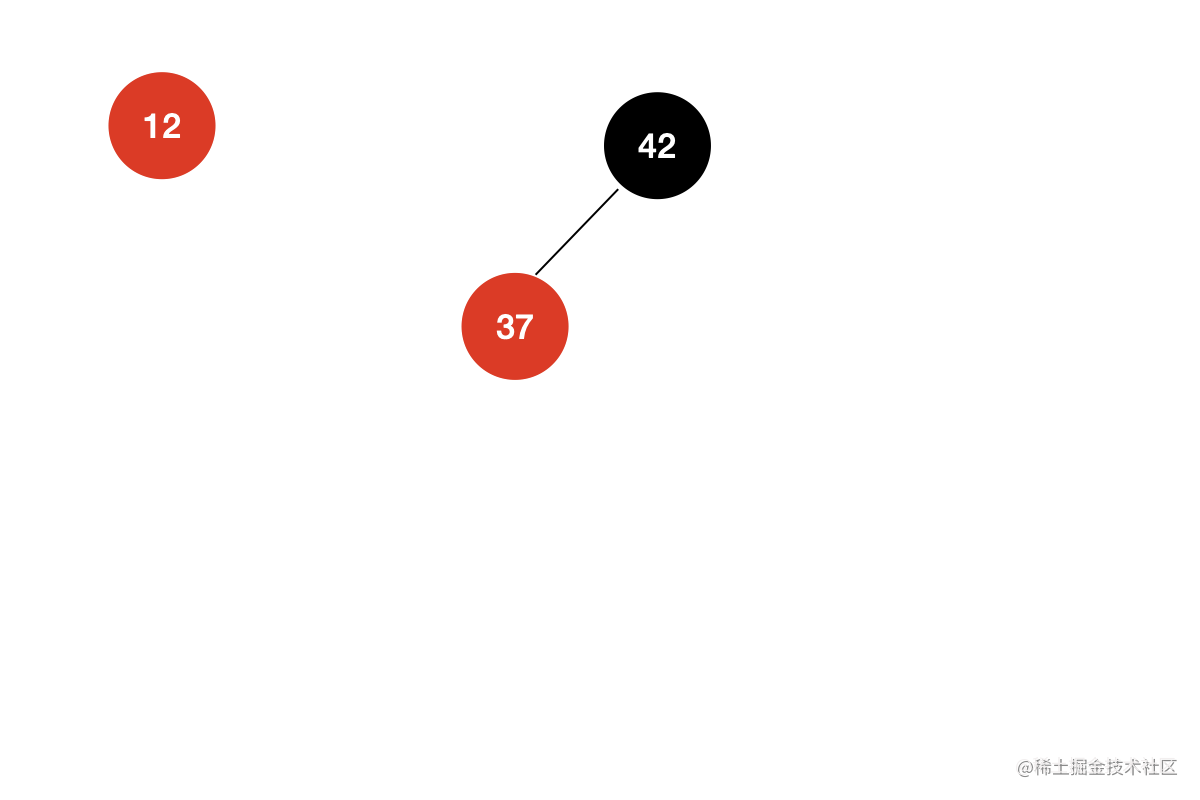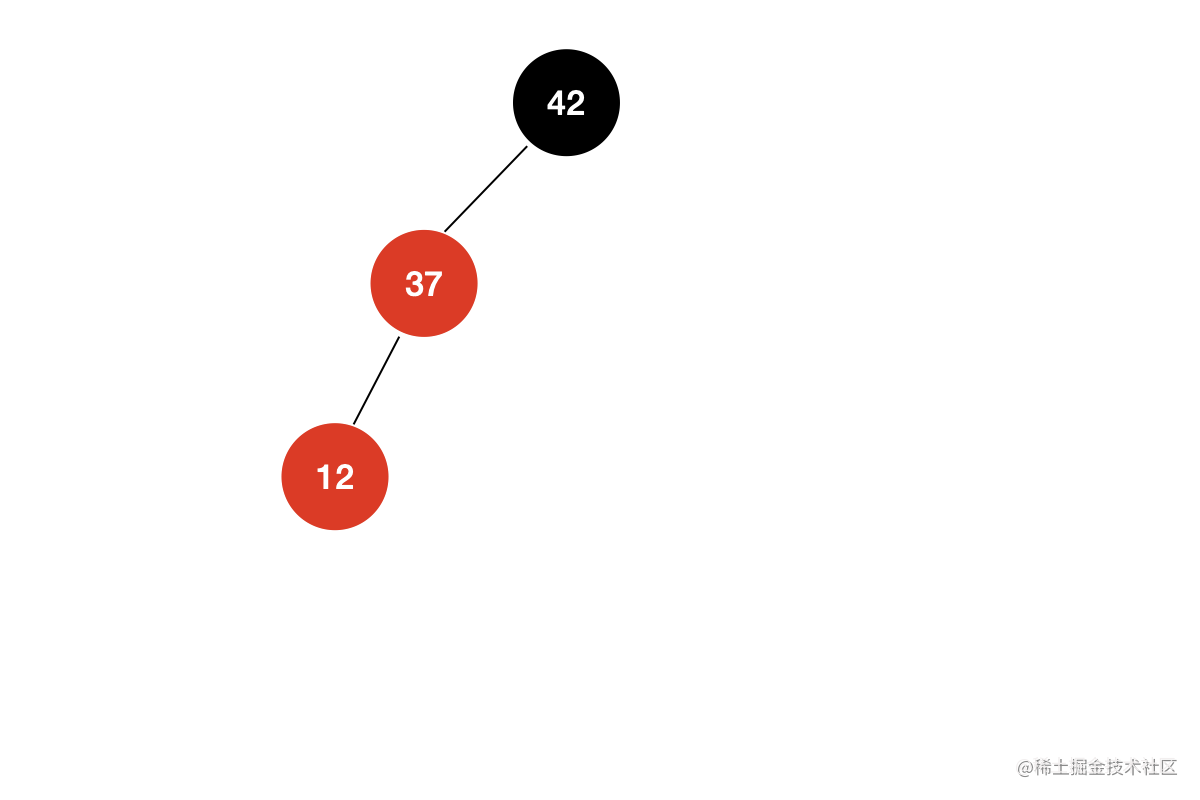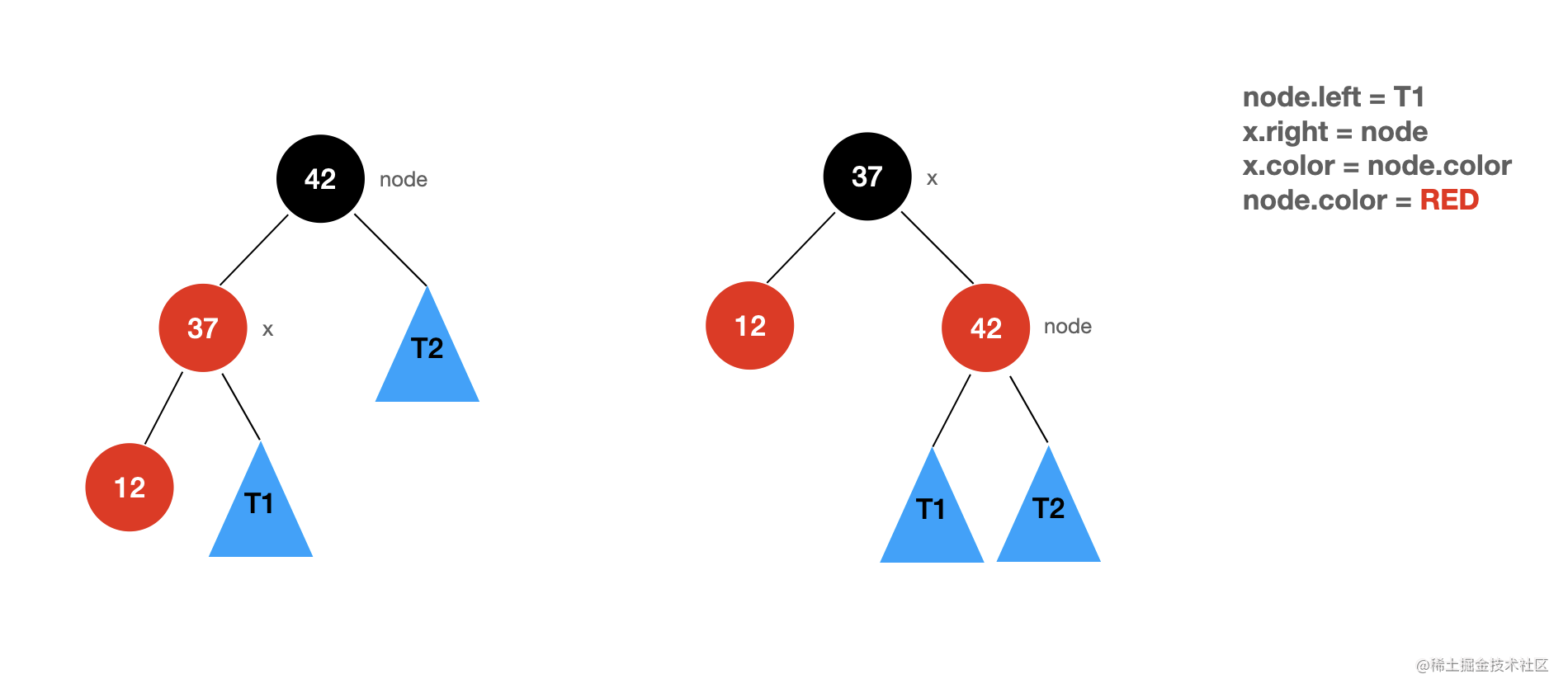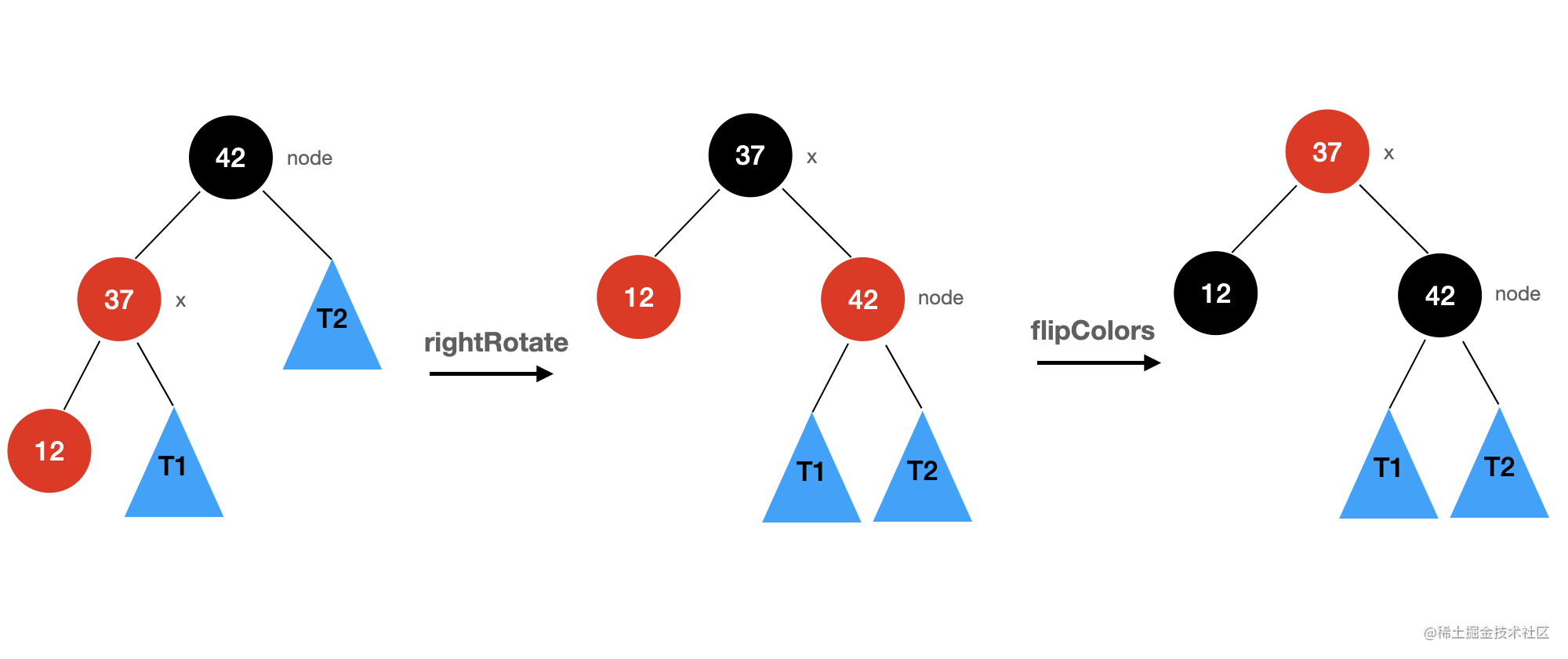/**
* 右旋转:
*
*        node                       x
*       /   \                     /  \
*     x      T2    ===========>  y   node
*   /  \                            /   \
*  y   T1                          T1   T2
*
*/
private Node rightRotate(Node node) {
Node x = node.left;

// 右旋转
node.left = x.right;
x.right = node;

x.color = node.color;
node.color = RED;
return x;
}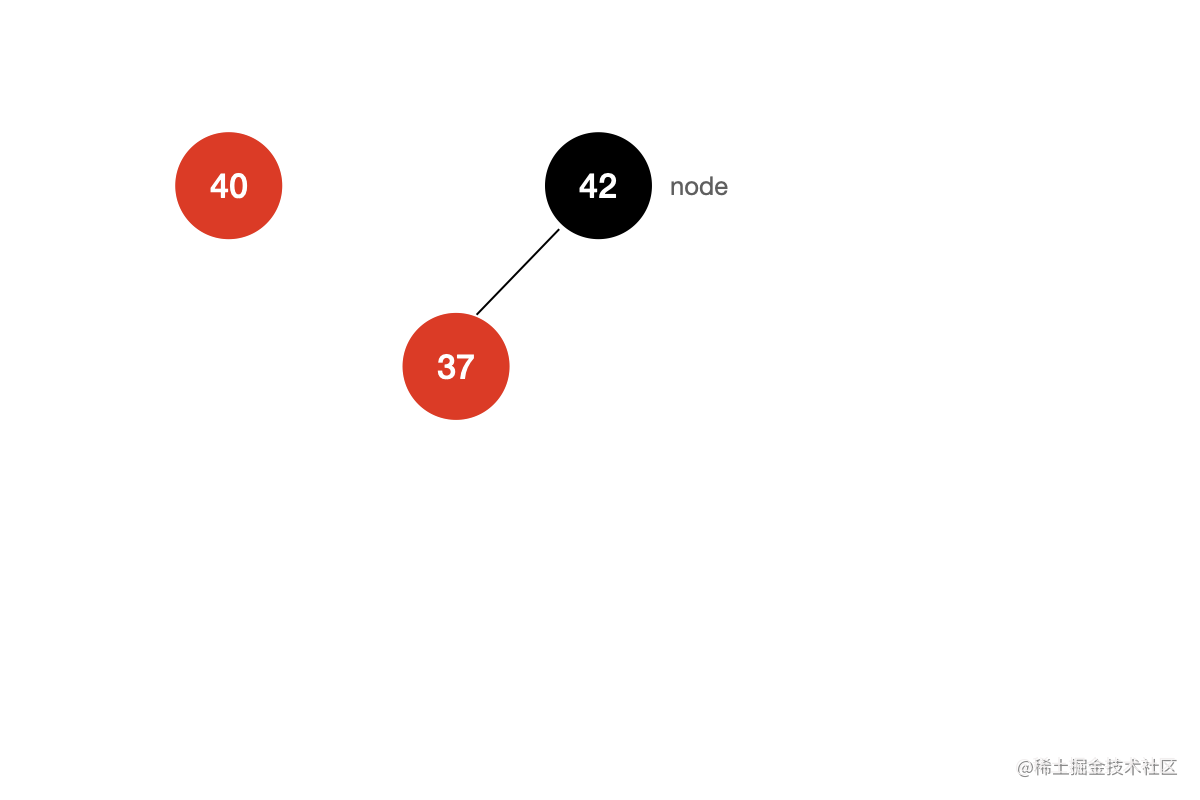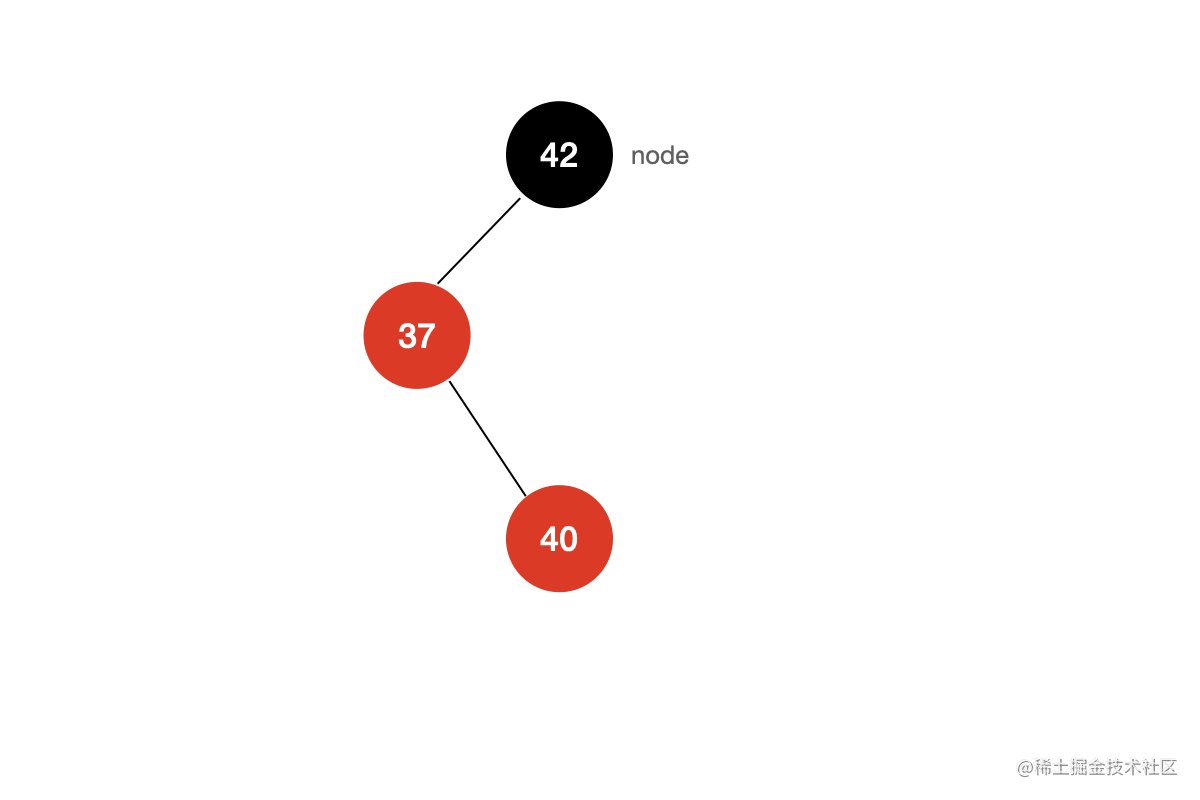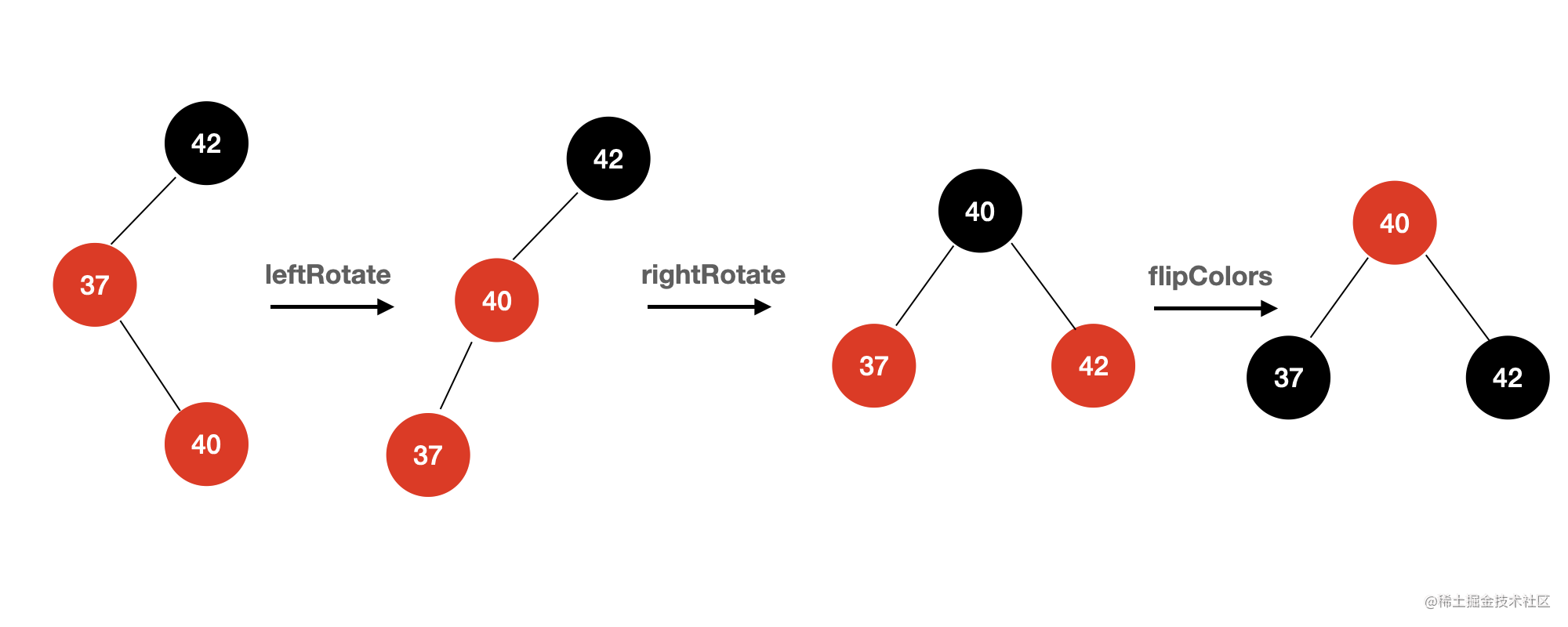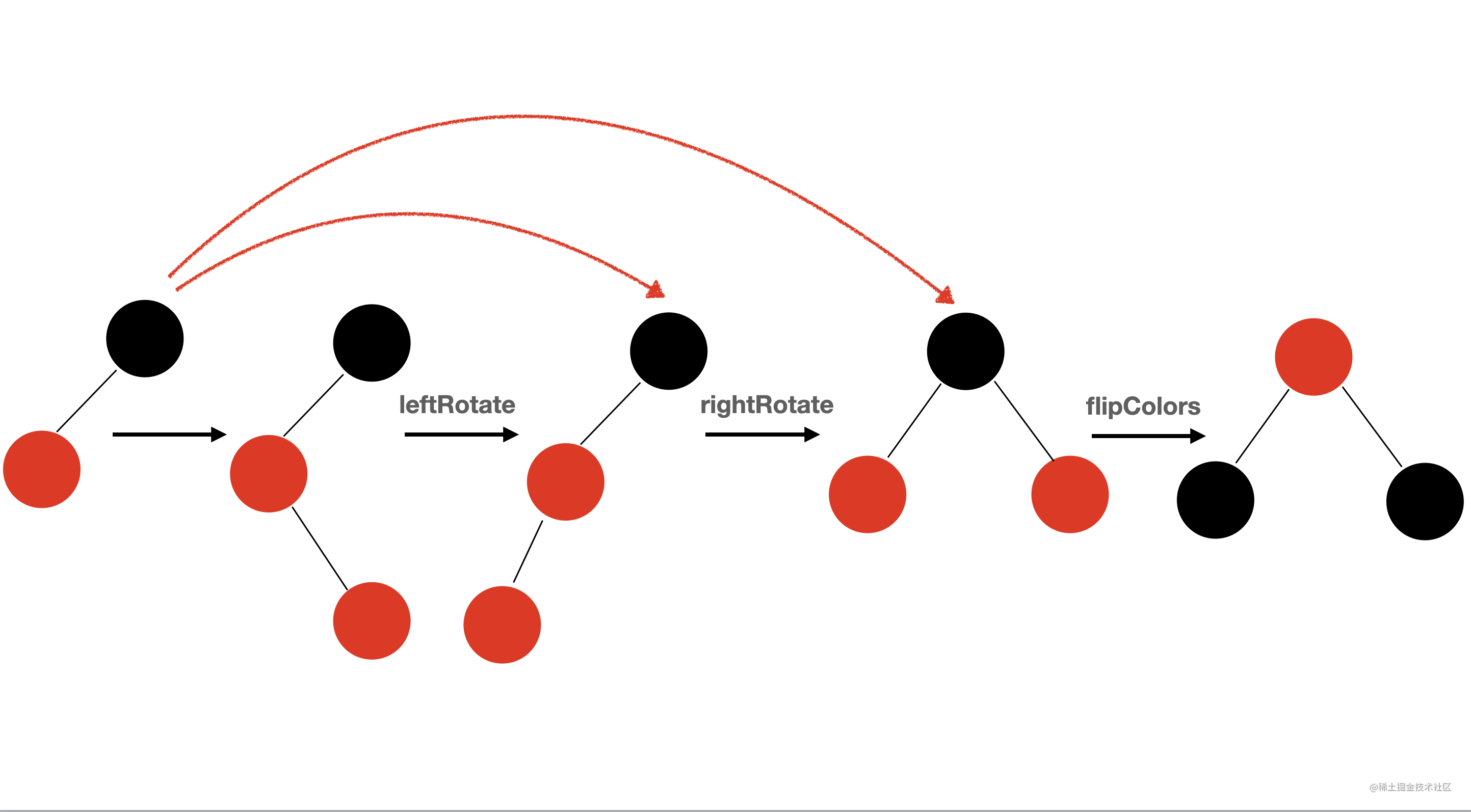public class RBTree<E extends Comparable<E>> {

private static final boolean RED = true;
private static final boolean BLACK = false;

private Node root;
private int size;

public RBTree() {
root = null;
size = 0;
}

public int size() {
return size;
}

public boolean isEmpty() {
return size == 0;
}

/**
* 判断节点 node 的颜色
*
* @param node
* @return
*/
public boolean isRed(Node node) {
if (node == null)
return false;
return node.color;
}

/**
* @return 返回红黑树的根节点
*/
public Node getRoot() {
return root;
}

/**
* 左旋转:
*
*        node                      x
*       /   \                    /  \
*     T1     x    ===========> node T3
*          /  \               /   \
*         T2  T3             T1   T2
*
*/
private Node leftRotate(Node node){
Node x = node.right;

// 左旋转
node.right = x.left;
x.left = node;

x.color = node.color;
node.color = RED;

return x;
}

/**
* 右旋转:
*
*        node                       x
*       /   \                     /  \
*     x      T2    ===========>  y   node
*   /  \                            /   \
*  y   T1                          T1   T2
*
*/
private Node rightRotate(Node node) {
Node x = node.left;

// 右旋转
node.left = x.right;
x.right = node;

x.color = node.color;
node.color = RED;
return x;
}

// 颜色翻转
private void flipColors(Node node){
node.color = RED;
node.left.color = BLACK;
node.right.color = BLACK;
}

/**
* @param e 向红黑树中添加新的元素
*/
root.color = BLACK;
}

/**
* @param e    向红黑树中新插入的节点
* @param node 当前比较的节点
* @return 返回红黑树的根节点
*/
private Node add(E e, Node node) {
if (node == null) {
size++;
return new Node(e); // 默认插入的是红色节点
}
if (e.compareTo((E) node.e) < 0) {
} else if (e.compareTo((E) node.e) > 0) {
}

if(isRed(node.right) && !isRed(node.left))
node = leftRotate(node);
if(isRed(node.left) && isRed(node.left.left))
rightRotate(node);
if(isRed(node.left) && isRed(node.right))
flipColors(node);
return node;
}
}

## 总结

1. 每个节点或者为红色，或者为黑色
2. 根节点的颜色一定是黑色
3. 每一个叶子节点（最后的空节点）一定是黑色的
4. 如果一个节点是红色的，那么它的孩子节点都是黑色的
5. 从任意一个节点到叶子节点，经过的黑色节点的数量一样多